# NCERT Exemplar Class 10 Maths Solutions for Chapter 6 - Triangles

## NCERT Exemplar Solutions Class 10 Maths Chapter 6 – Free PDF Download

NCERT Exemplar Class 10 Maths Chapter 6 Triangles, provided here along with solutions by our subject experts to make students get prepared for the board exams with respect to CBSE Syllabus (2021-2022). Students can download the PDF file and use it as an effective study-tool to practice math problems and also have a quick revision of the topics.

Triangle and its properties are a familiar topic for Class 10 students as they must have studied about it in earlier classes. However, we all know that the concepts get more advanced and new topics are introduced as we progress through each class. As such, in Class 10, the topic of triangles is explained more in detail and students have to deal with new and more complex sums as well as concepts like Pythagoras theorem, the similarity of triangles, similar figures, and more.

The topics covered in these exemplar problems and solutions are:

• Similar figures
• Triangles and their similarity
• Conditions for similarity in triangles
• Areas of similar triangles and their ratios
• Triangle theorems and proofs based on Pythagoras theorem

Check out Class 10 Maths Chapter 6 NCERT Exemplar PDF below.

## Download PDF of NCERT Exemplar Solutions for Class 10 Maths Chapter 6 Triangles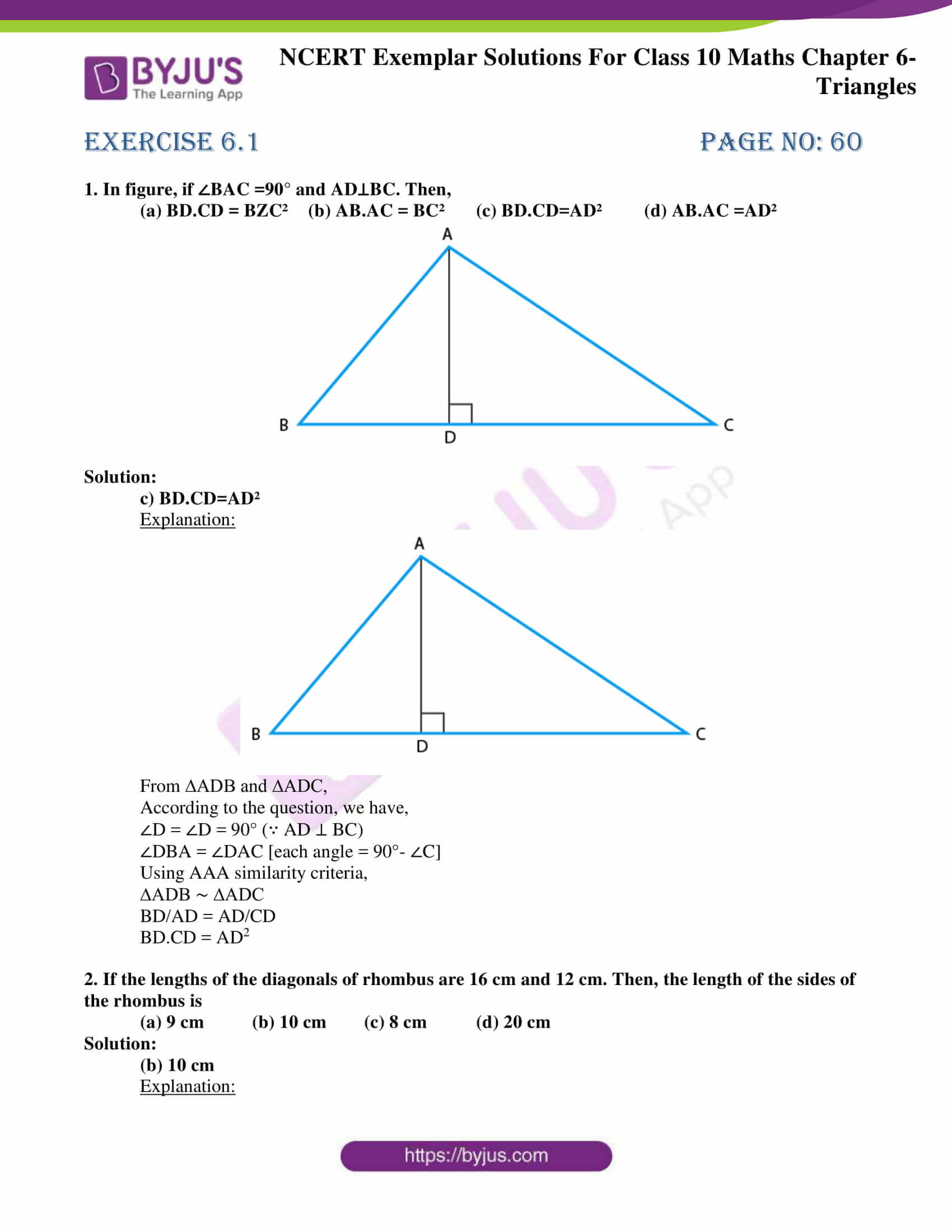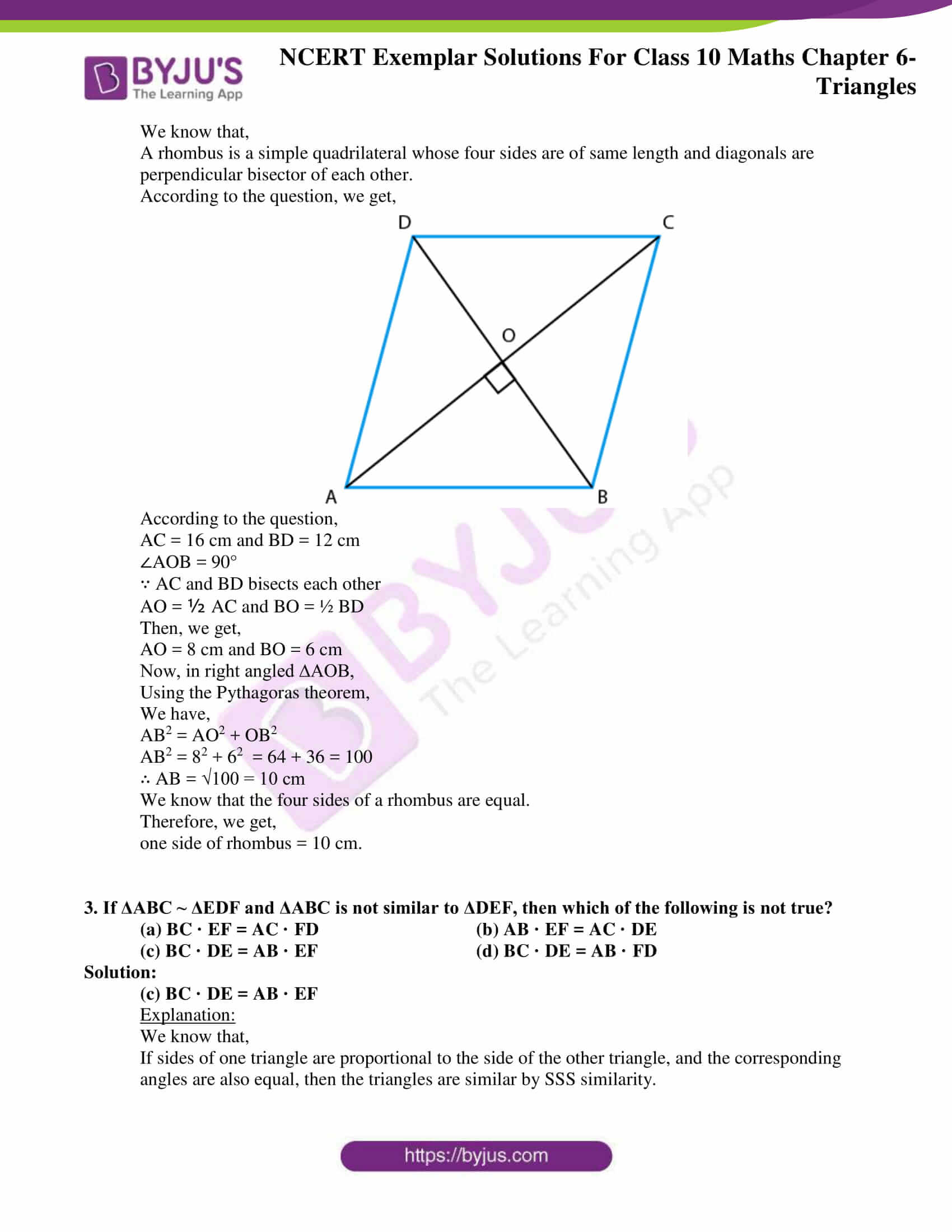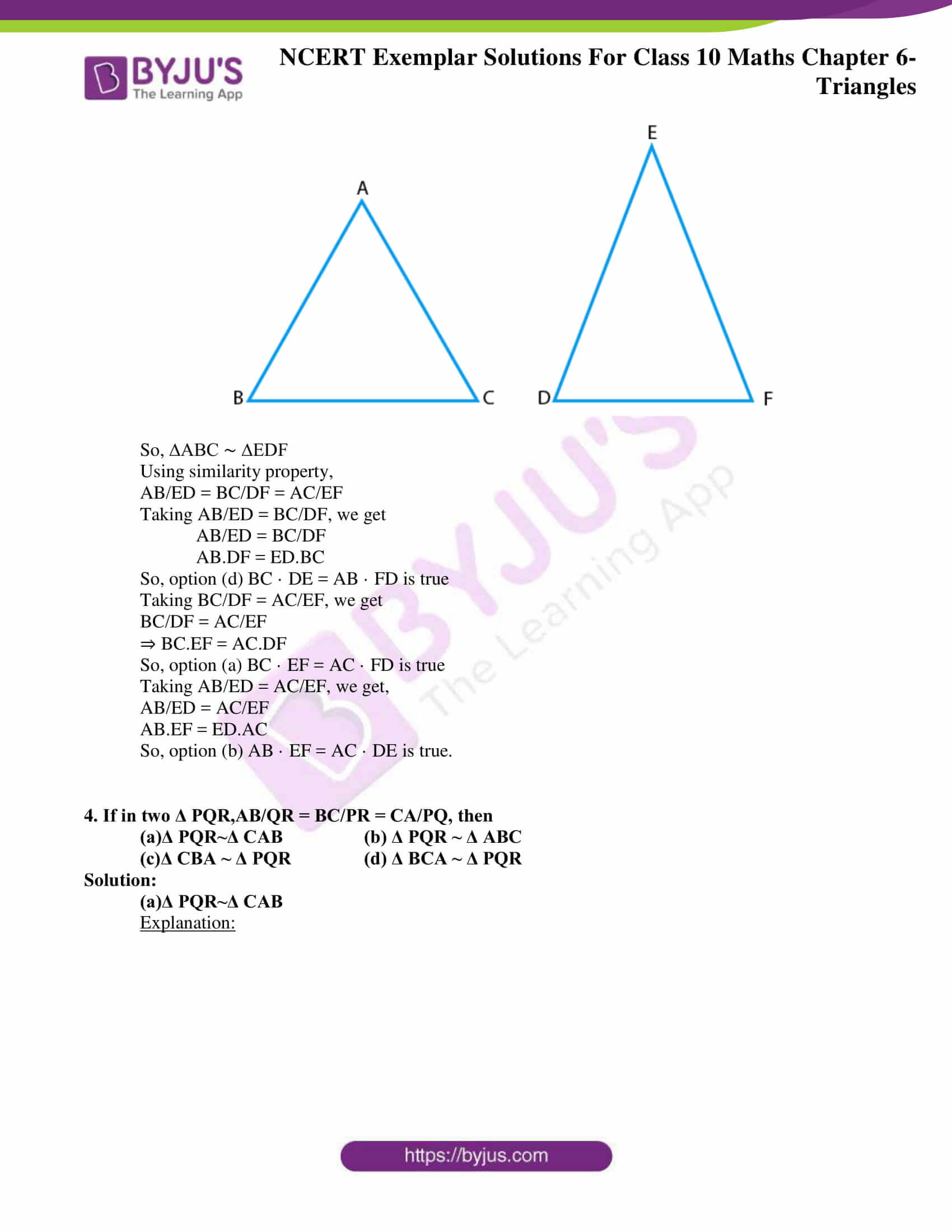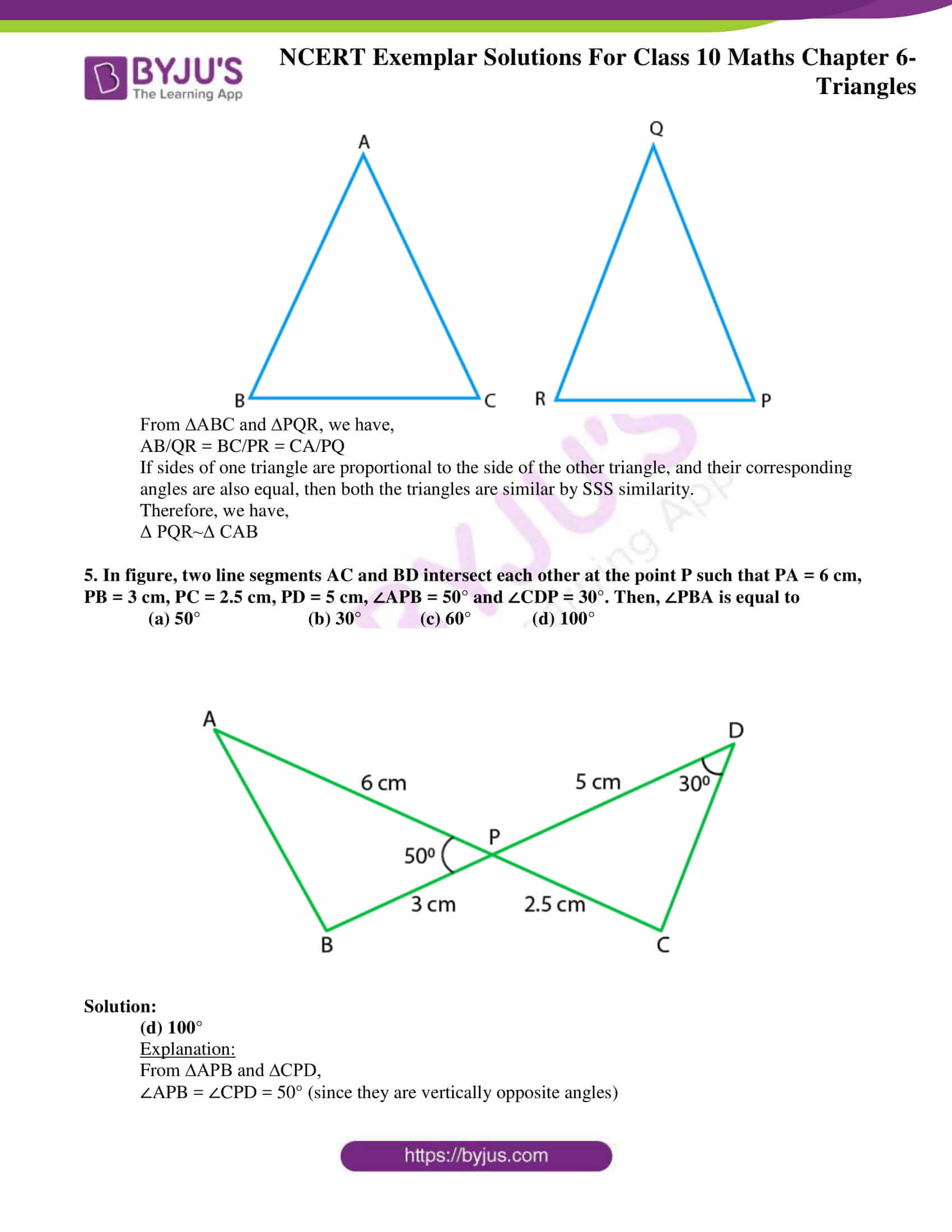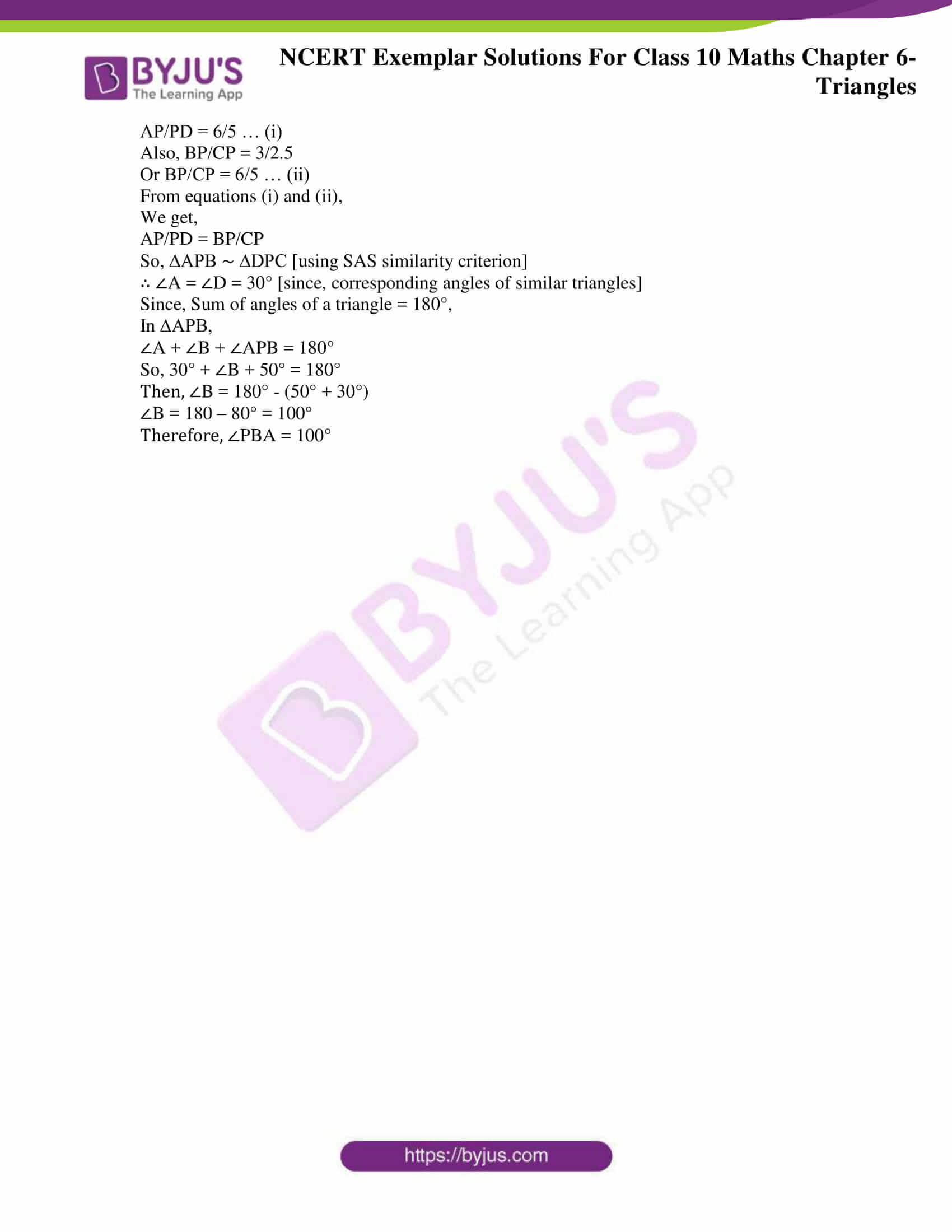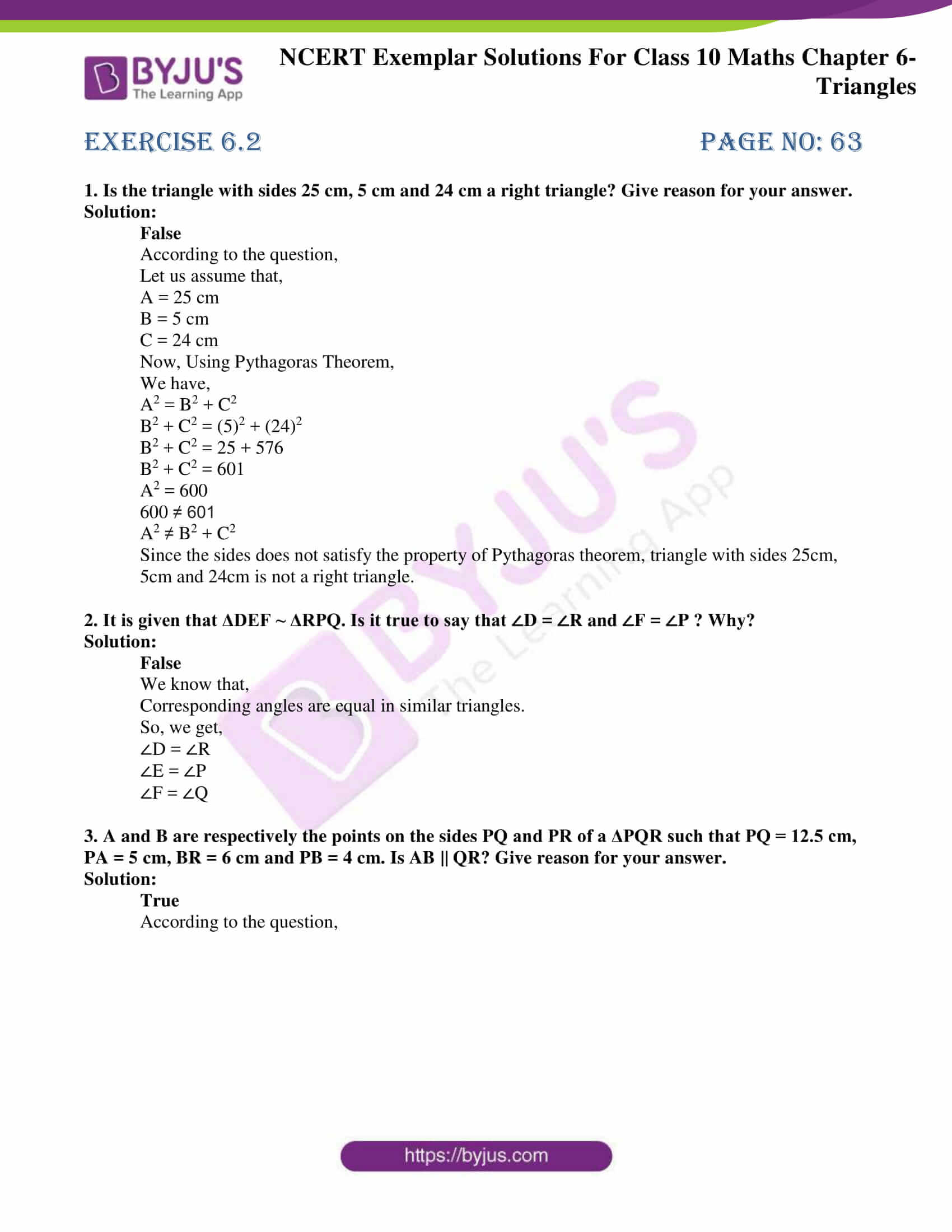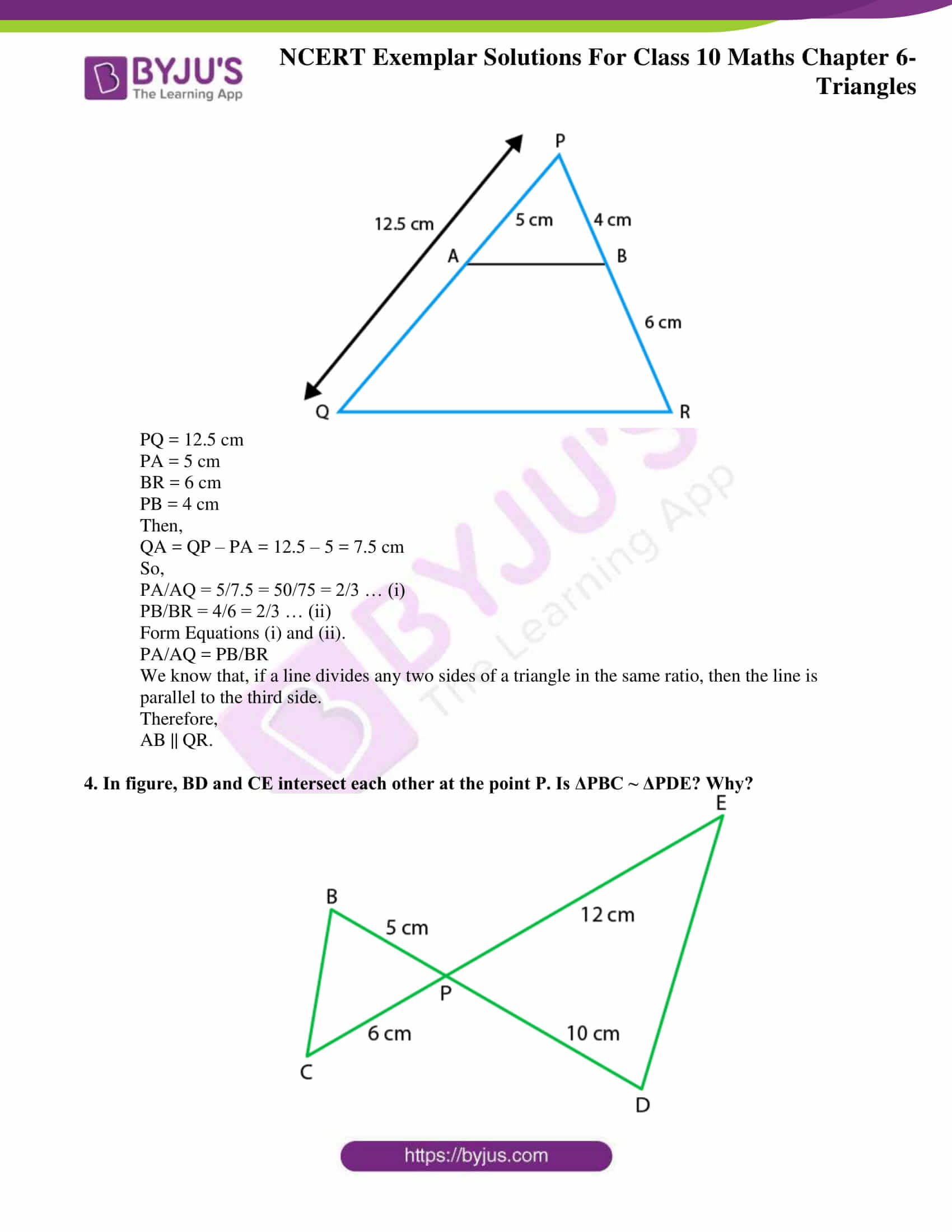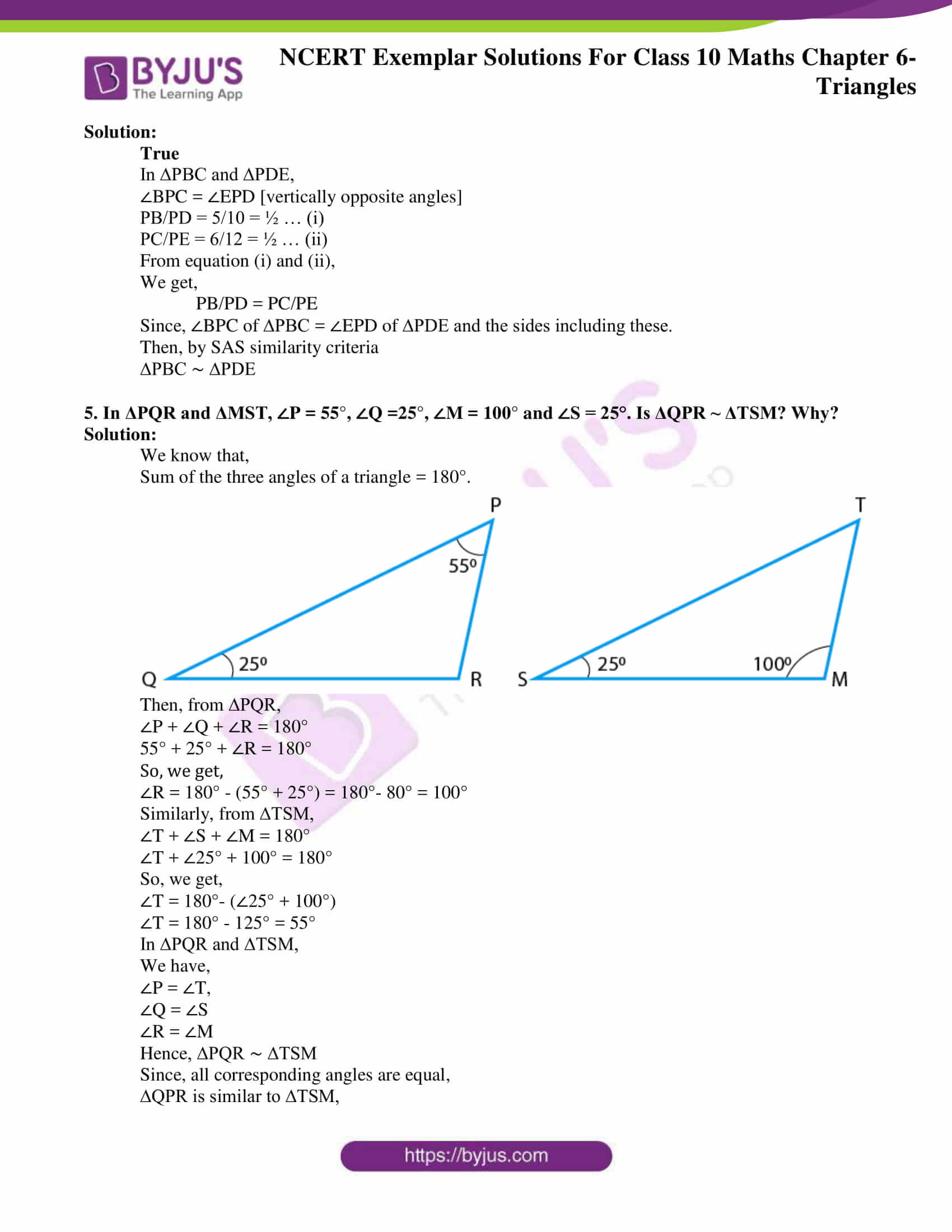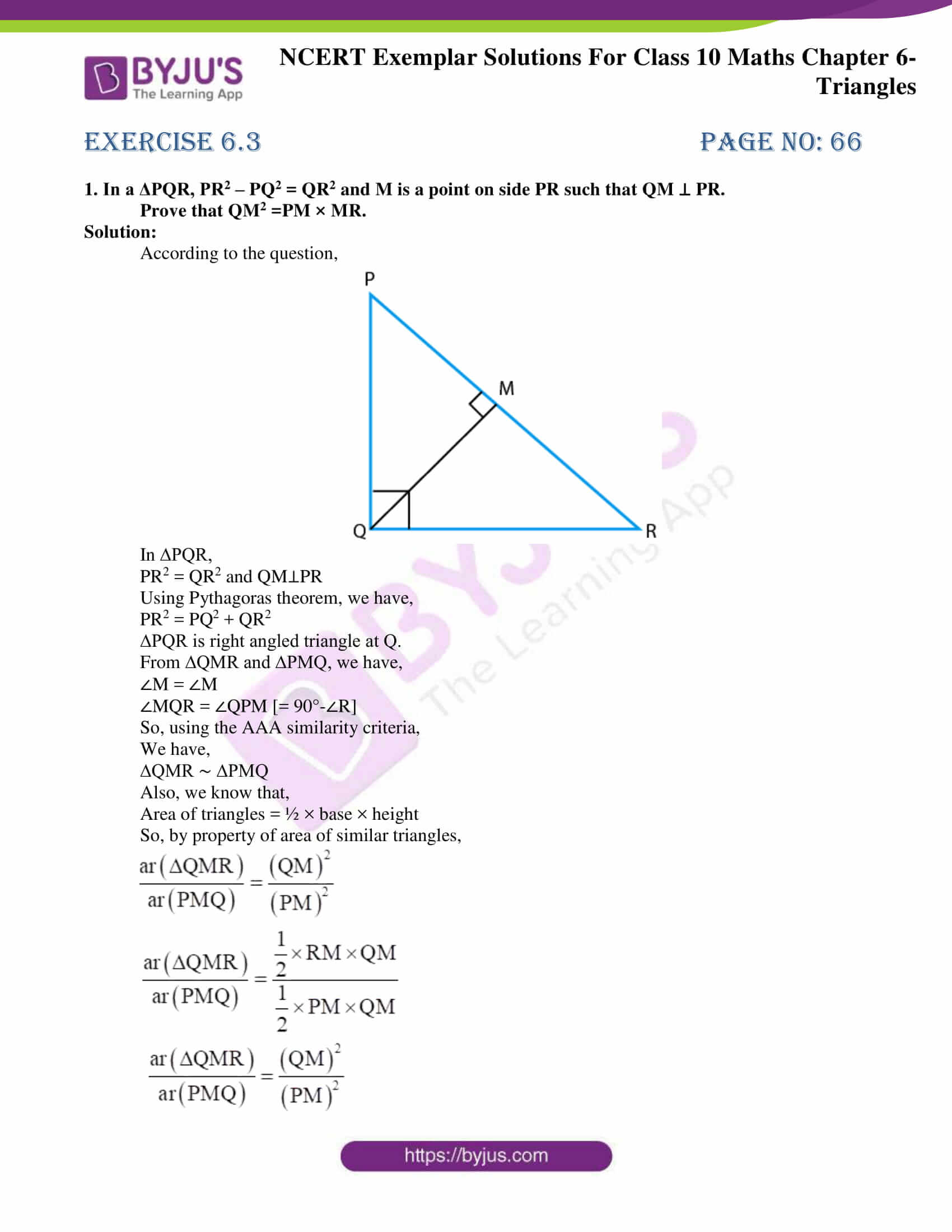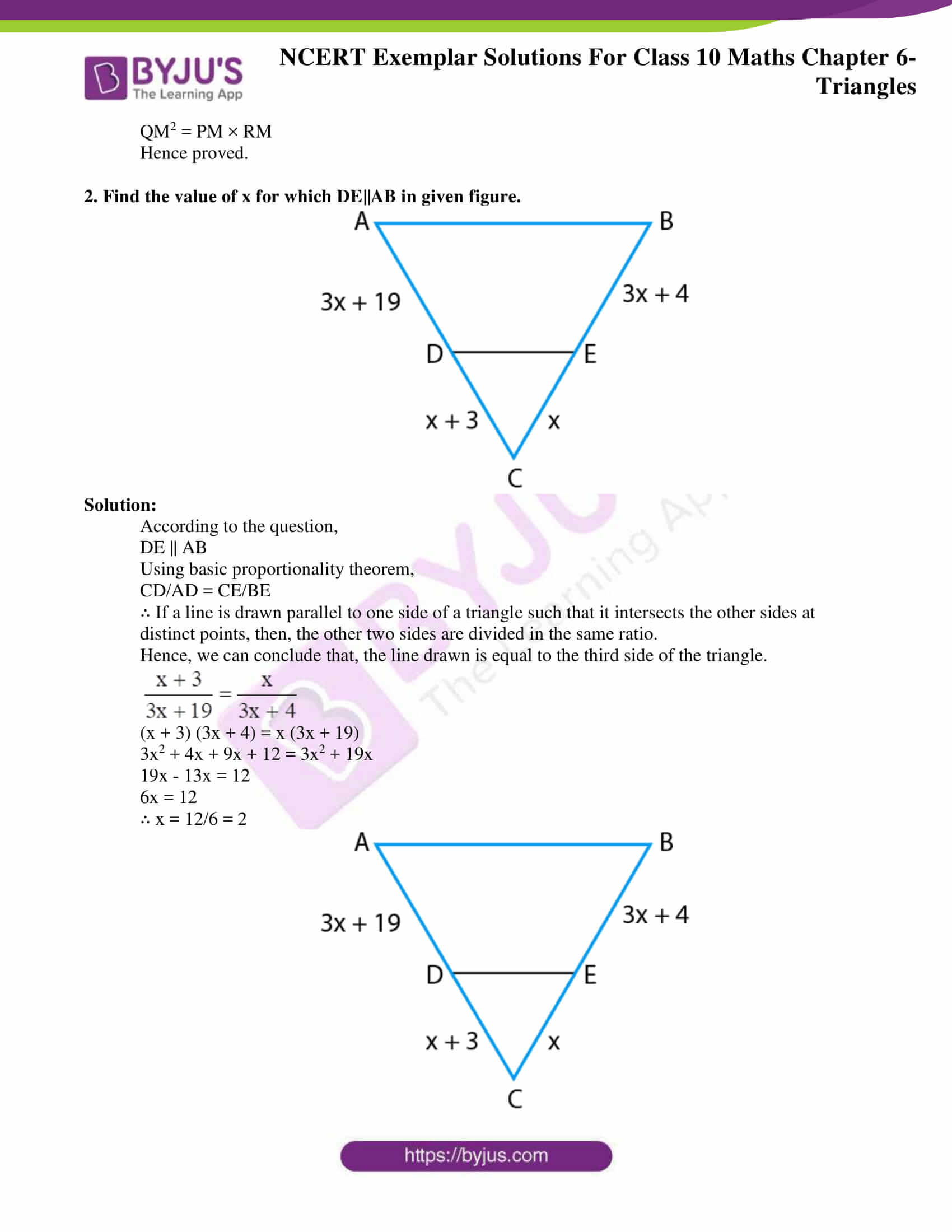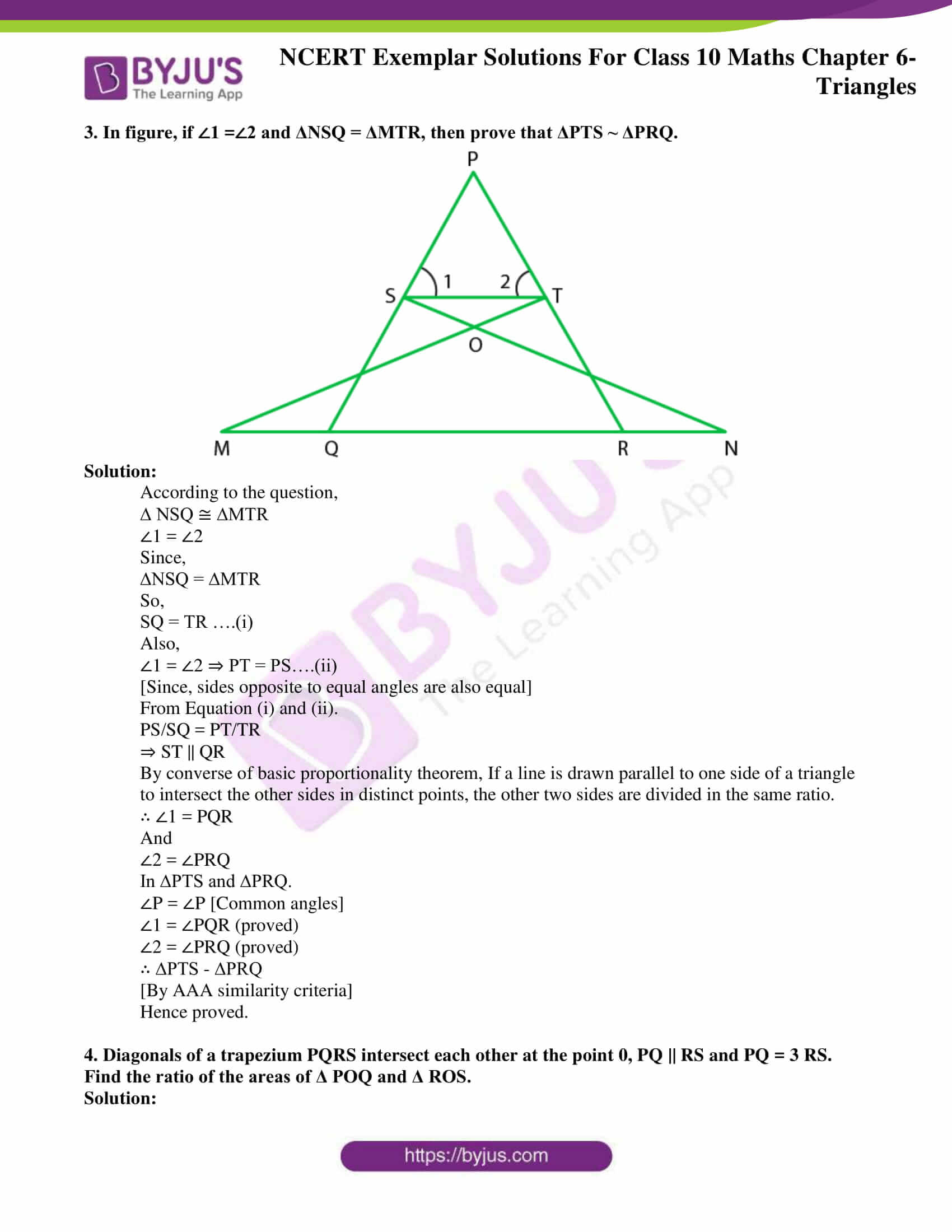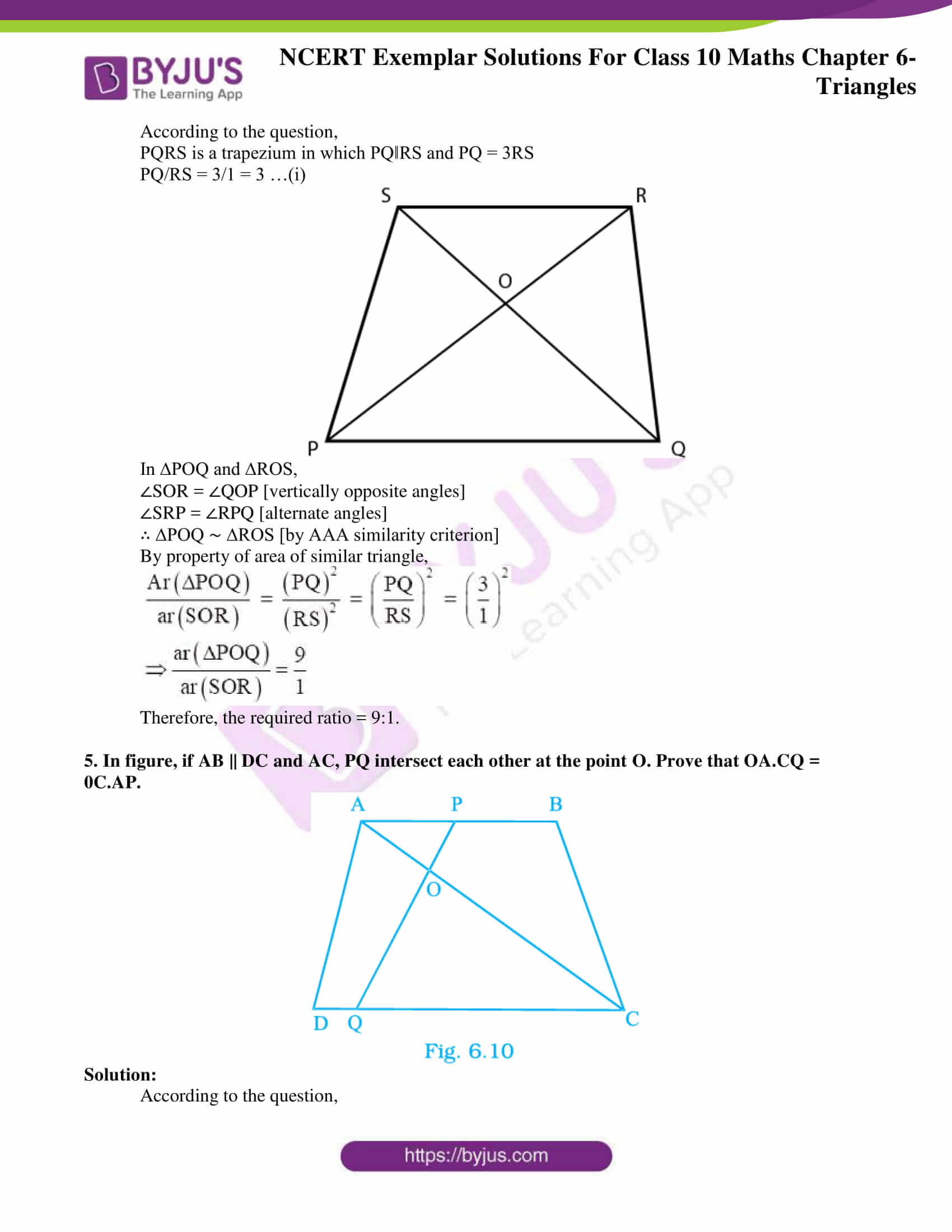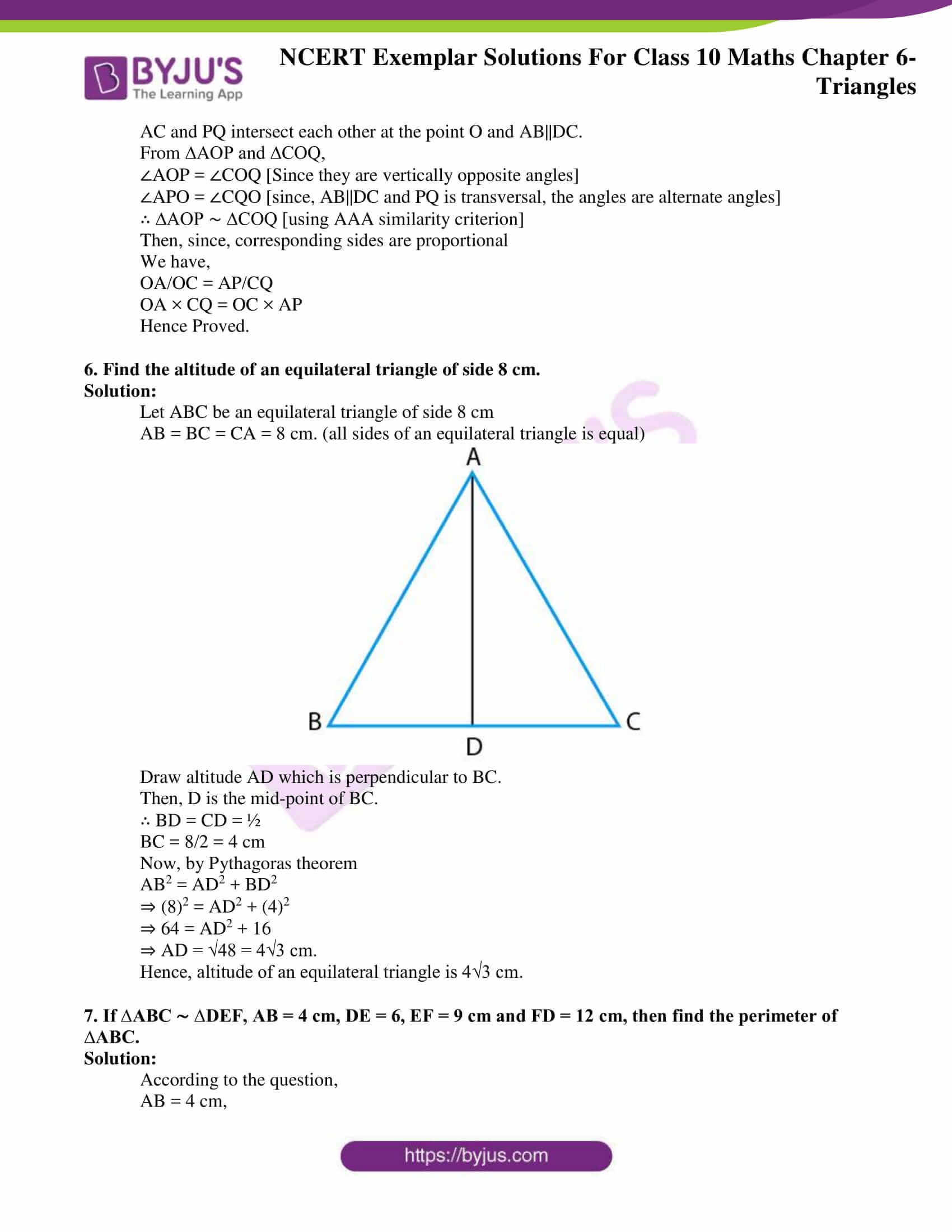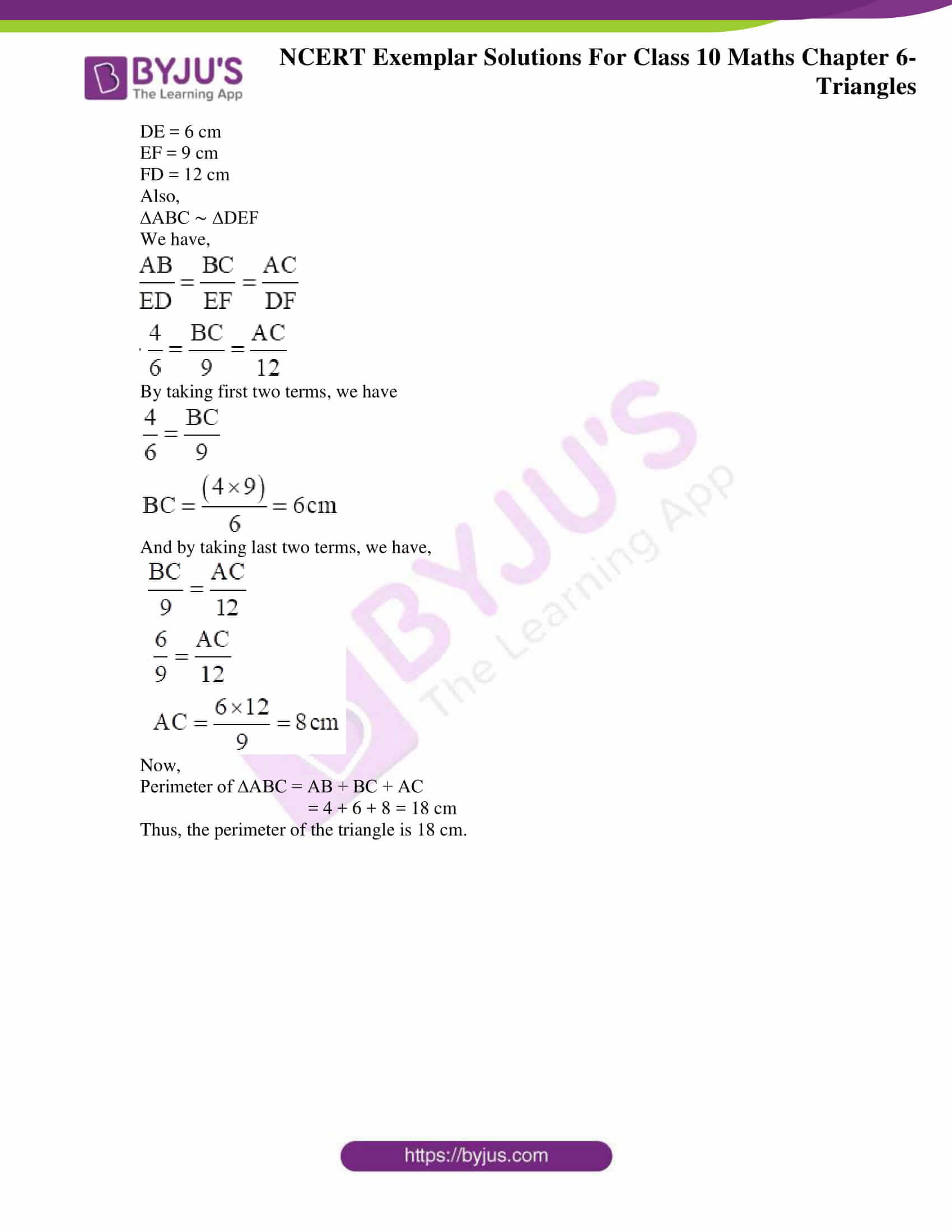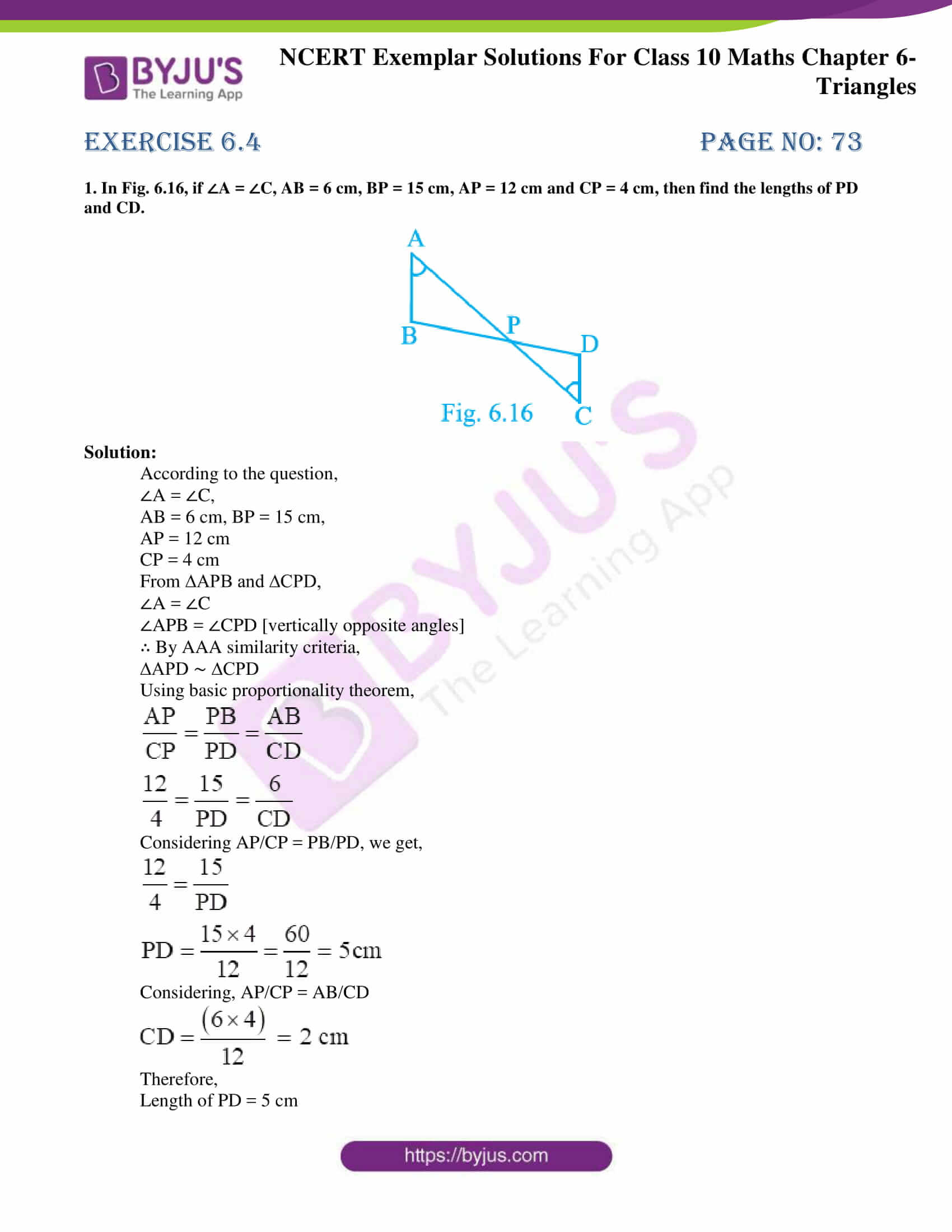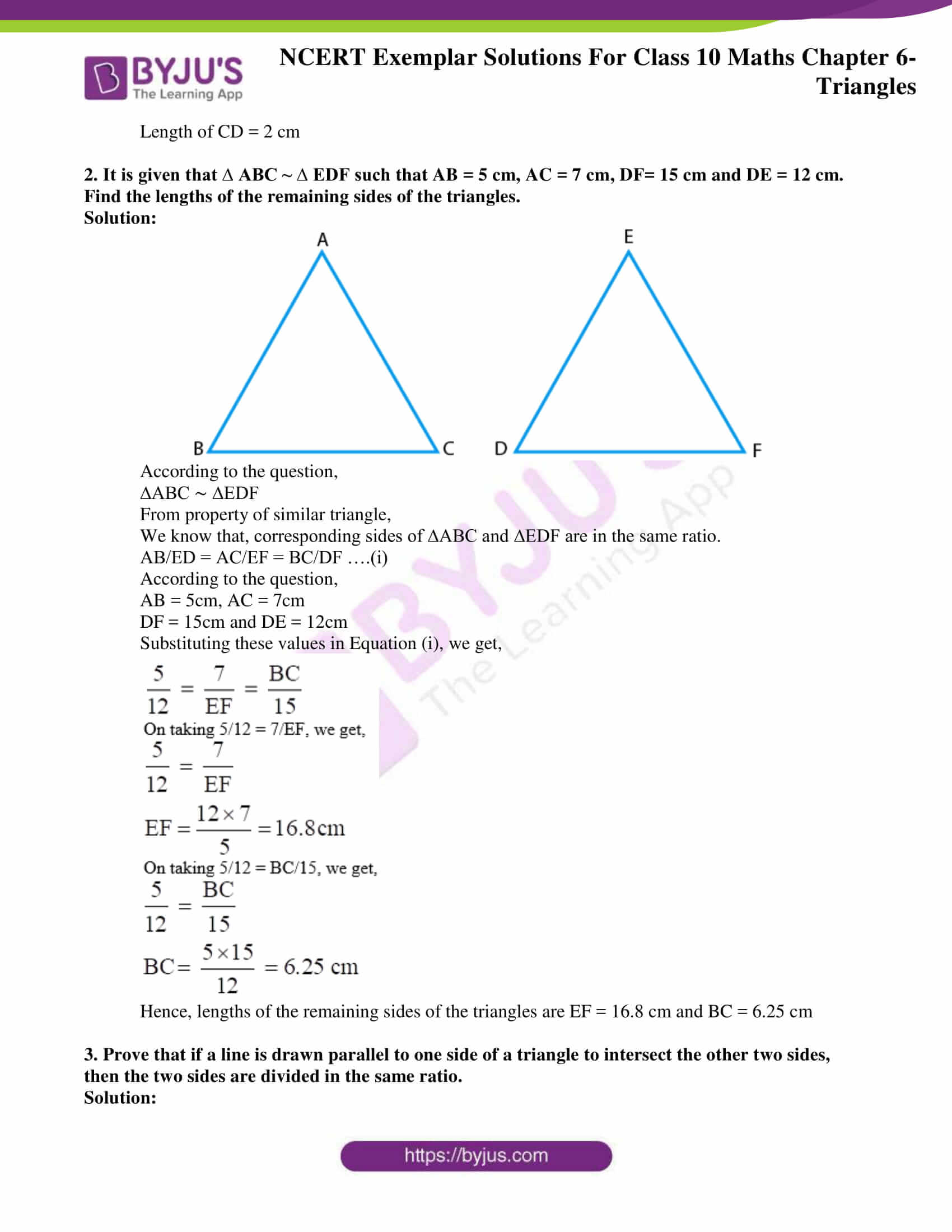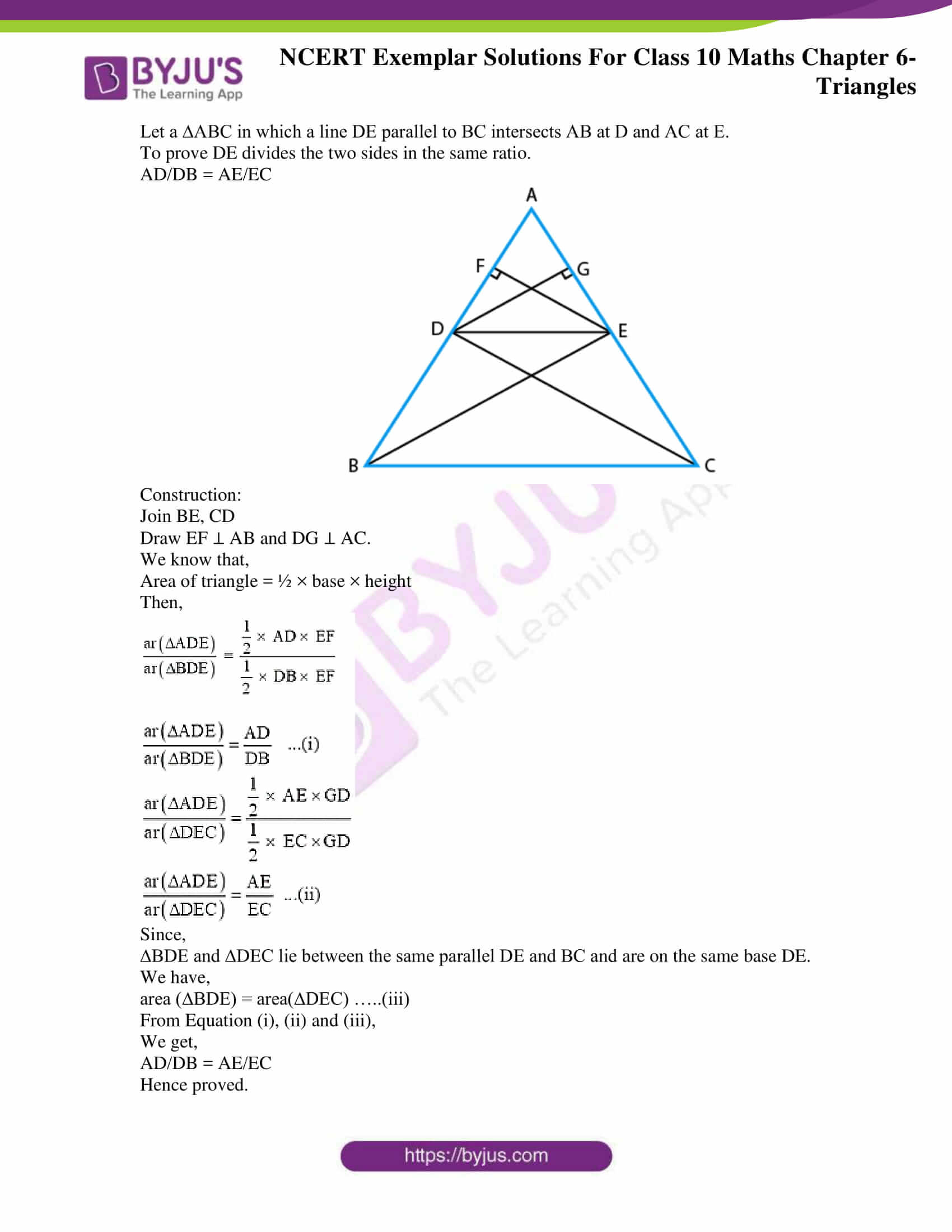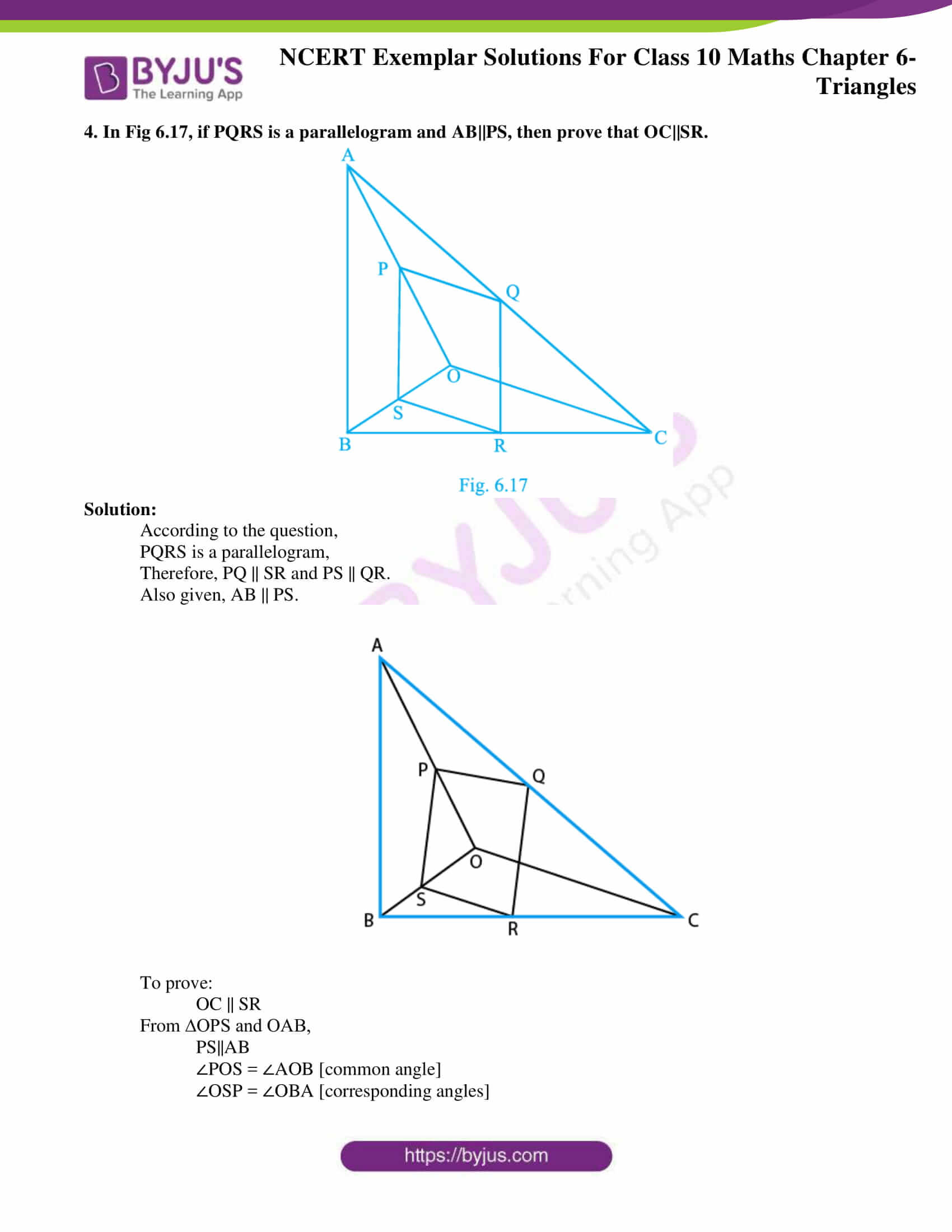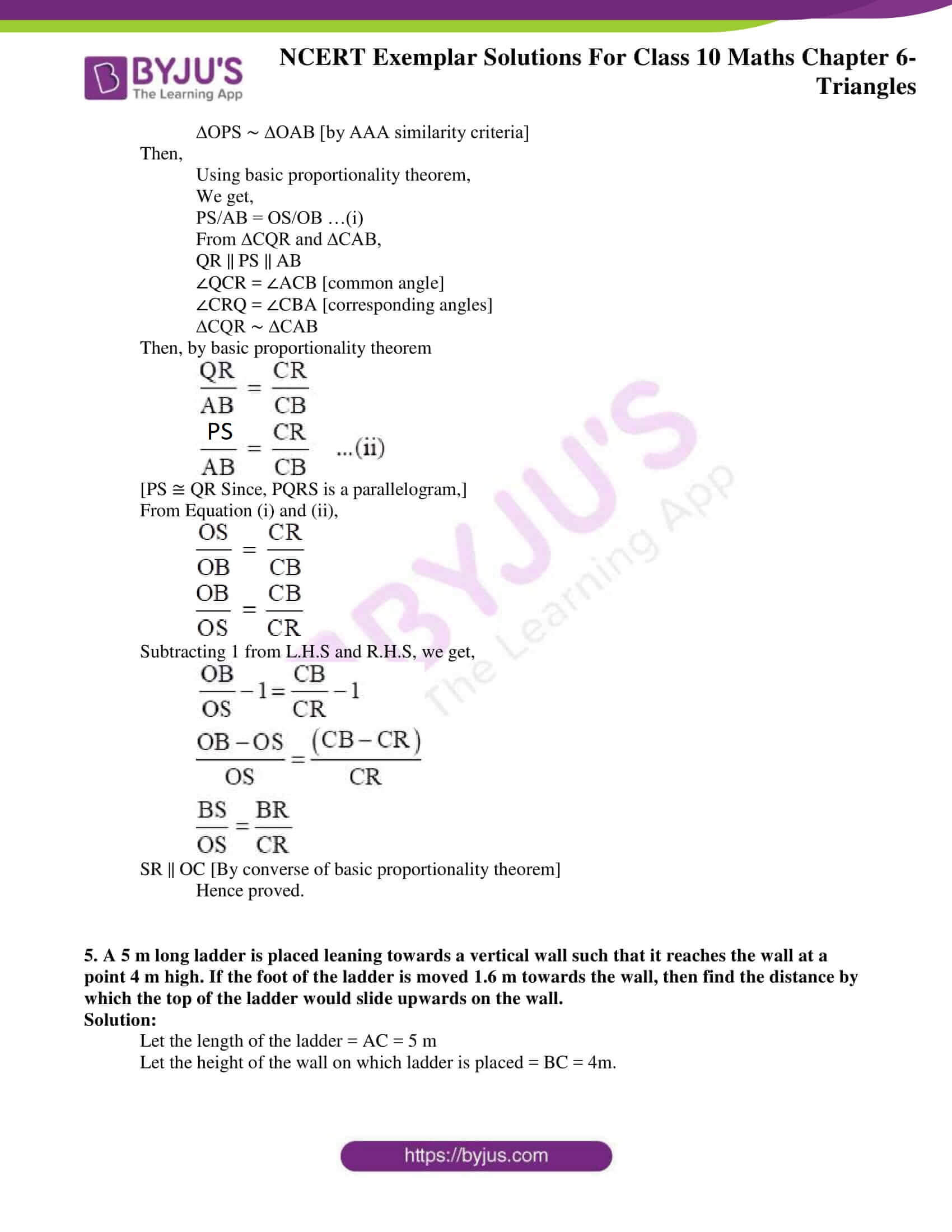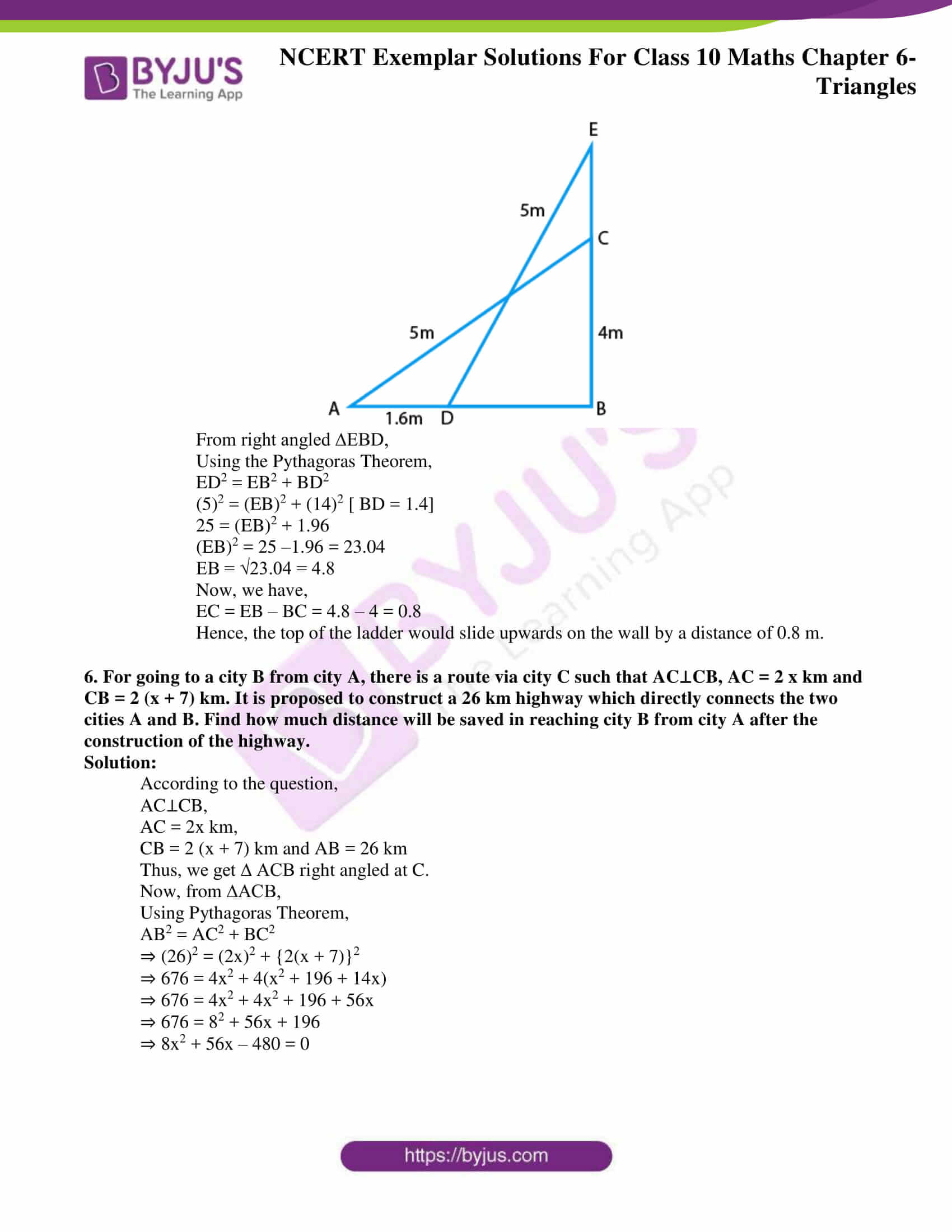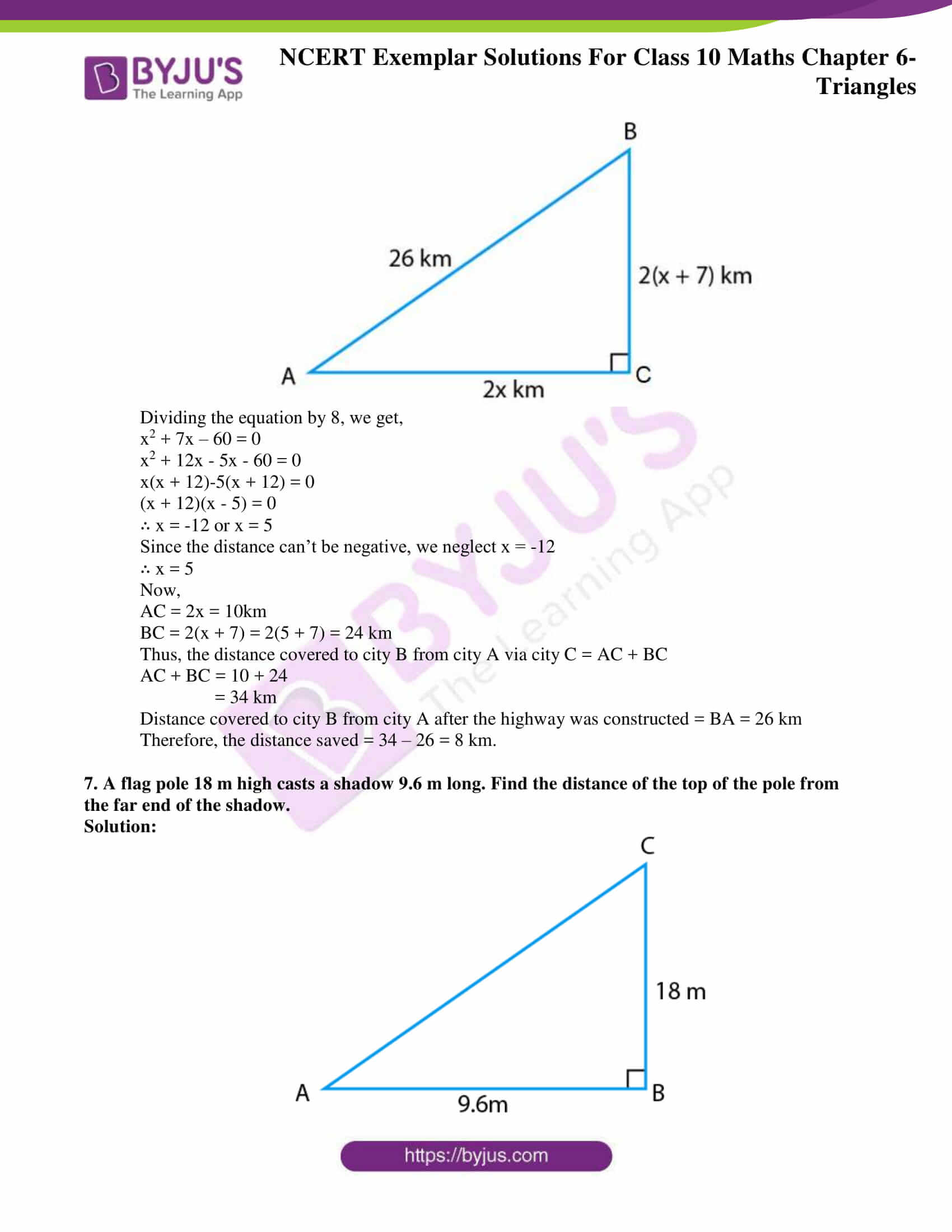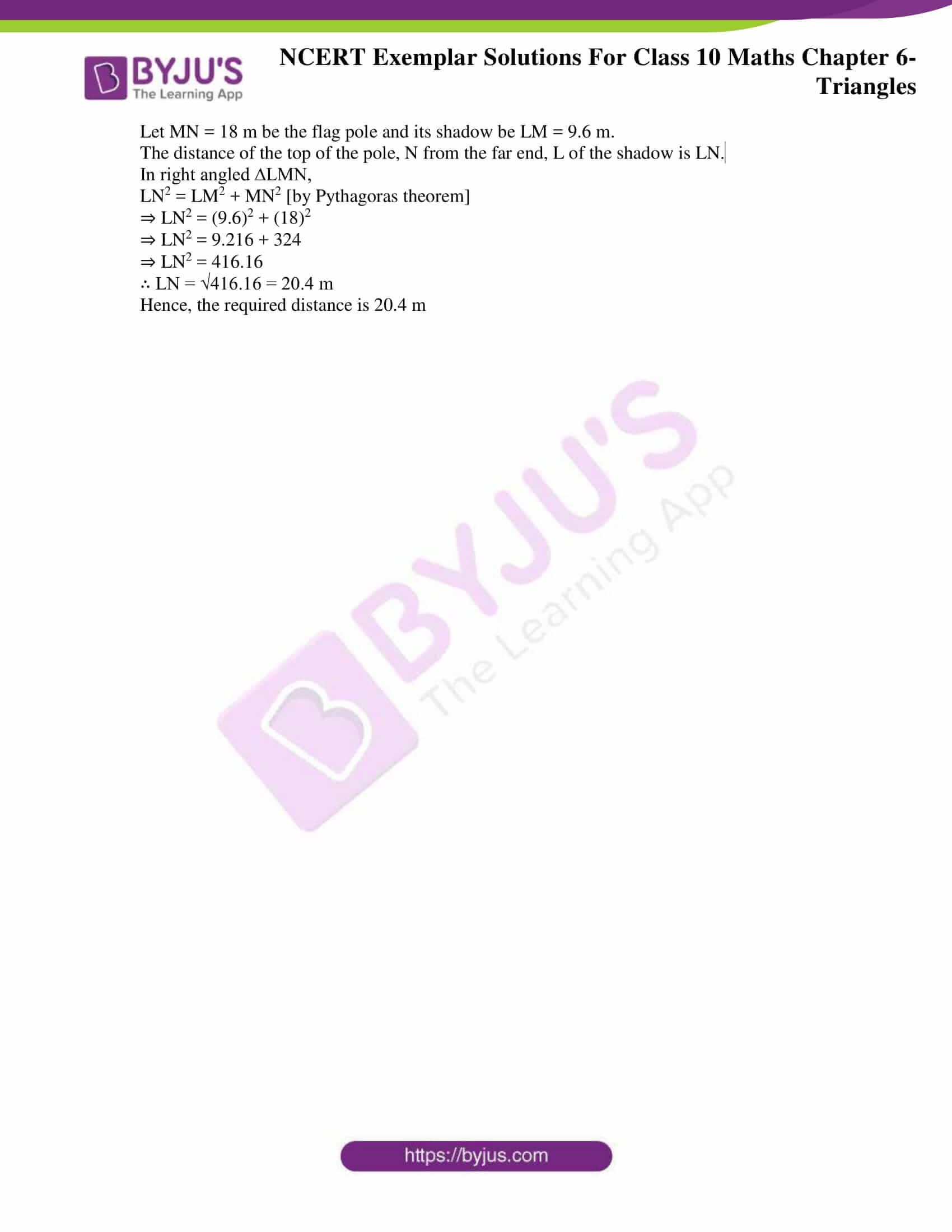## Exercise 6.1

1. In figure, if ∠BAC =90° and AD⊥BC. Then,

(a) BD.CD = BZC² (b) AB.AC = BC² (c) BD.CD=AD² (d) AB.AC =AD²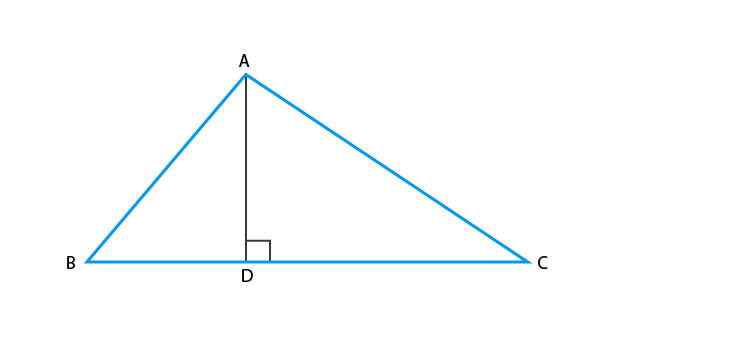Solution:

Explanation:According to the question, we have,

∠D = ∠D = 90° (∵ AD ⊥ BC)

∠DBA = ∠DAC [each angle = 90°- ∠C]

Using AAA similarity criteria,

2. If the lengths of the diagonals of rhombus are 16 cm and 12 cm. Then, the length of the sides of the rhombus is

(a) 9 cm (b) 10 cm (c) 8 cm (d) 20 cm

Solution:

(b) 10 cm

Explanation:

We know that,

A rhombus is a simple quadrilateral whose four sides are of same length and diagonals are perpendicular bisector of each other.

According to the question, we get,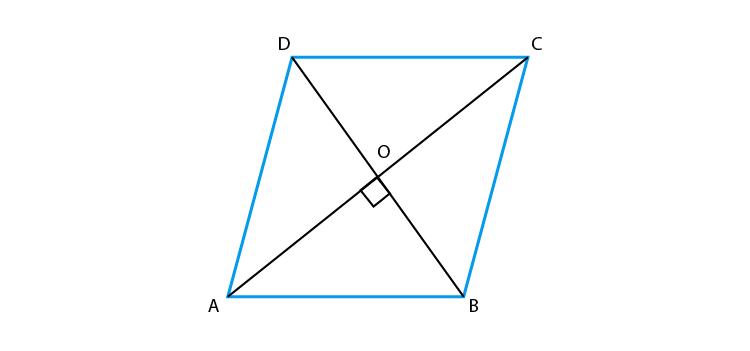According to the question,

AC = 16 cm and BD = 12 cm

∠AOB = 90°

∵ AC and BD bisects each other

AO = ½ AC and BO = ½ BD

Then, we get,

AO = 8 cm and BO = 6 cm

Now, in right angled ∆AOB,

Using the Pythagoras theorem,

We have,

AB2 = AO2 + OB2

AB2 = 82 + 62 = 64 + 36 = 100

∴ AB = √100 = 10 cm

We know that the four sides of a rhombus are equal.

Therefore, we get,

one side of rhombus = 10 cm.

3. If ΔABC ~ ΔEDF and ΔABC is not similar to ΔDEF, then which of the following is not true?

(a) BC · EF = AC · FD (b) AB · EF = AC · DE

(c) BC · DE = AB · EF (d) BC · DE = AB · FD

Solution:

(c) BC · DE = AB · EF

Explanation:

We know that,

If sides of one triangle are proportional to the side of the other triangle, and the corresponding angles are also equal, then the triangles are similar by SSS similarity.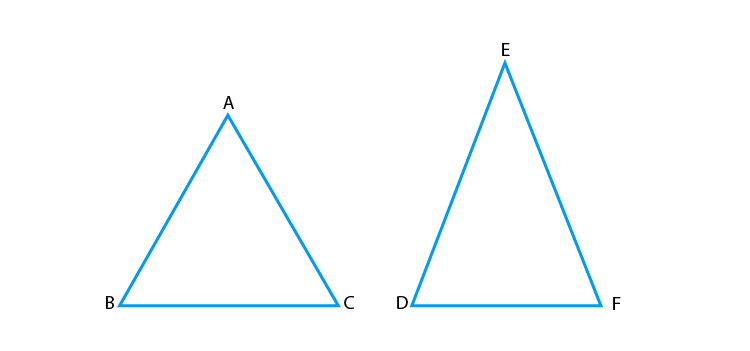So, ∆ABC ∼ ∆EDF

Using similarity property,

AB/ED = BC/DF = AC/EF

Taking AB/ED = BC/DF, we get

AB/ED = BC/DF

AB.DF = ED.BC

So, option (d) BC · DE = AB · FD is true

Taking BC/DF = AC/EF, we get

BC/DF = AC/EF

⇒ BC.EF = AC.DF

So, option (a) BC · EF = AC · FD is true

Taking AB/ED = AC/EF, we get,

AB/ED = AC/EF

AB.EF = ED.AC

So, option (b) AB · EF = AC · DE is true.

4. If in two Δ PQR,AB/QR = BC/PR = CA/PQ, then

(a)Δ PQR~Δ CAB (b) Δ PQR ~ Δ ABC

(c)Δ CBA ~ Δ PQR (d) Δ BCA ~ Δ PQR

Solution:

(a)Δ PQR~Δ CAB

Explanation: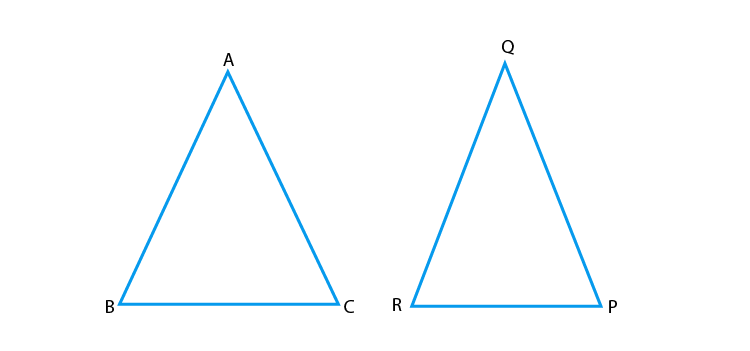From ∆ABC and ∆PQR, we have,

AB/QR = BC/PR = CA/PQ

If sides of one triangle are proportional to the side of the other triangle, and their corresponding angles are also equal, then both the triangles are similar by SSS similarity.

Therefore, we have,

Δ PQR~Δ CAB

5. In figure, two line segments AC and BD intersect each other at the point P such that PA = 6 cm, PB = 3 cm, PC = 2.5 cm, PD = 5 cm, ∠APB = 50° and ∠CDP = 30°. Then, ∠PBA is equal to

(a) 50° (b) 30° (c) 60° (d) 100°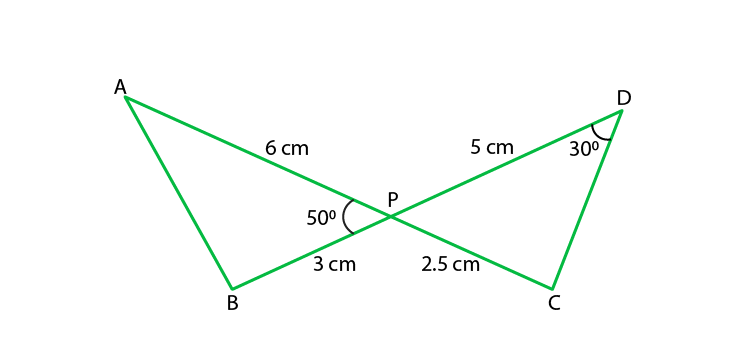Solution:

(d) 100°

Explanation:

From ∆APB and ∆CPD,

∠APB = ∠CPD = 50° (since they are vertically opposite angles)

AP/PD = 6/5 … (i)

Also, BP/CP = 3/2.5

Or BP/CP = 6/5 … (ii)

From equations (i) and (ii),

We get,

AP/PD = BP/CP

So, ∆APB ∼ ∆DPC [using SAS similarity criterion]

∴ ∠A = ∠D = 30° [since, corresponding angles of similar triangles]

Since, Sum of angles of a triangle = 180°,

In ∆APB,

∠A + ∠B + ∠APB = 180°

So, 30° + ∠B + 50° = 180°

Then, ∠B = 180° – (50° + 30°)

∠B = 180 – 80° = 100°

Therefore, ∠PBA = 100°

## Exercise 6.2

1. Is the triangle with sides 25 cm, 5 cm and 24 cm a right triangle? Give reason for your answer.

Solution:

False

According to the question,

Let us assume that,

A = 25 cm

B = 5 cm

C = 24 cm

Now, Using Pythagoras Theorem,

We have,

A2 = B2 + C2

B2 + C2 = (5)2 + (24)2

B2 + C2 = 25 + 576

B2 + C2 = 601

A2 = 600

600 ≠ 601

A2 ≠ B2 + C2

Since the sides does not satisfy the property of Pythagoras theorem, triangle with sides 25cm, 5cm and 24cm is not a right triangle.

2. It is given that ΔDEF ~ ΔRPQ. Is it true to say that ∠D = ∠R and ∠F = ∠P ? Why?

Solution:

False

We know that,

Corresponding angles are equal in similar triangles.

So, we get,

∠D = ∠R

∠E = ∠P

∠F = ∠Q

3. A and B are respectively the points on the sides PQ and PR of a ΔPQR such that PQ = 12.5 cm, PA = 5 cm, BR = 6 cm and PB = 4 cm. Is AB || QR? Give reason for your answer.

Solution:

True

According to the question,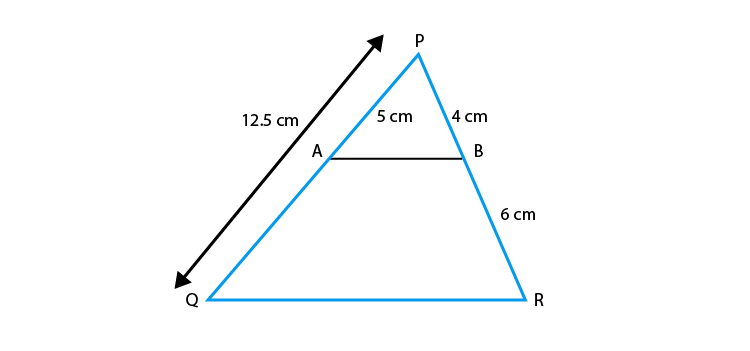PQ = 12.5 cm

PA = 5 cm

BR = 6 cm

PB = 4 cm

Then,

QA = QP – PA = 12.5 – 5 = 7.5 cm

So,

PA/AQ = 5/7.5 = 50/75 = 2/3 … (i)

PB/BR = 4/6 = 2/3 … (ii)

Form Equations (i) and (ii).

PA/AQ = PB/BR

We know that, if a line divides any two sides of a triangle in the same ratio, then the line is parallel to the third side.

Therefore,

AB || QR.

4. In figure, BD and CE intersect each other at the point P. Is ΔPBC ~ ΔPDE? Why?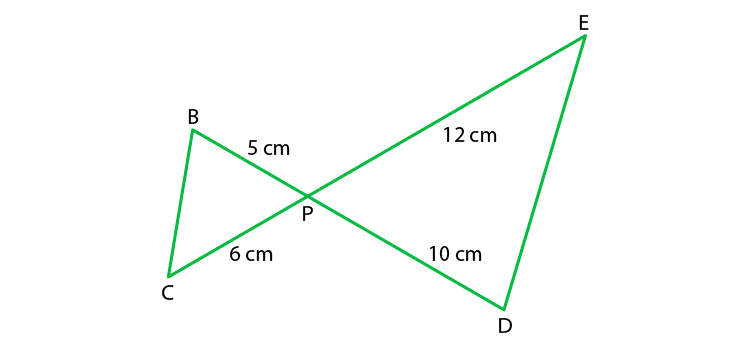Solution:

True

In ∆PBC and ∆PDE,

∠BPC = ∠EPD [vertically opposite angles]

PB/PD = 5/10 = ½ … (i)

PC/PE = 6/12 = ½ … (ii)

From equation (i) and (ii),

We get,

PB/PD = PC/PE

Since, ∠BPC of ∆PBC = ∠EPD of ∆PDE and the sides including these.

Then, by SAS similarity criteria

∆PBC ∼ ∆PDE

5. In ΔPQR and ΔMST, ∠P = 55°, ∠Q =25°, ∠M = 100° and ∠S = 25°. Is ΔQPR ~ ΔTSM? Why?

Solution:

We know that,

Sum of the three angles of a triangle = 180°.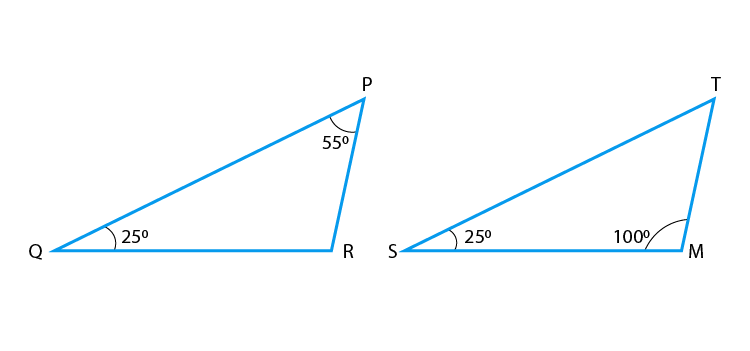Then, from ∆PQR,

∠P + ∠Q + ∠R = 180°

55° + 25° + ∠R = 180°

So, we get,

∠R = 180° – (55° + 25°) = 180°- 80° = 100°

Similarly, from ∆TSM,

∠T + ∠S + ∠M = 180°

∠T + ∠25° + 100° = 180°

So, we get,

∠T = 180°- (∠25° + 100°)

∠T = 180° – 125° = 55°

In ∆PQR and ∆TSM,

We have,

∠P = ∠T,

∠Q = ∠S

∠R = ∠M

Hence, ∆PQR ∼ ∆TSM

Since, all corresponding angles are equal,

∆QPR is similar to ∆TSM,

6. Is the following statement true? Why?

“Two quadrilaterals are similar, if their corresponding angles are equal”.

Solution:

False

Two quadrilaterals cannot be similar, if only their corresponding angles are equal

## Exercise 6.3

1. In a ΔPQR, PR2 – PQ2 = QR2 and M is a point on side PR such that QM ⊥ PR.

Prove that QM2 =PM × MR.

Solution:

According to the question,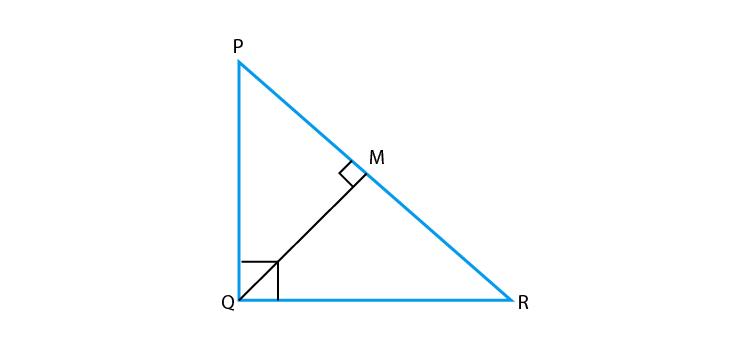In ∆PQR,

PR2 = QR2 and QM⊥PR

Using Pythagoras theorem, we have,

PR2 = PQ2 + QR2

∆PQR is right angled triangle at Q.

From ∆QMR and ∆PMQ, we have,

∠M = ∠M

∠MQR = ∠QPM [= 90°-∠R]

So, using the AAA similarity criteria,

We have,

∆QMR ∼ ∆PMQ

Also, we know that,

Area of triangles = ½ × base × height

So, by property of area of similar triangles,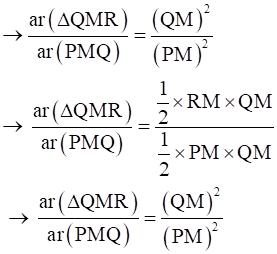QM2 = PM × RM

Hence proved.

2. Find the value of x for which DE||AB in given figure.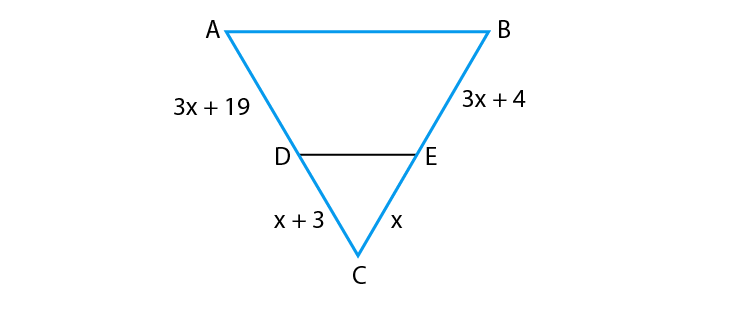Solution:

According to the question,

DE || AB

Using basic proportionality theorem,

∴ If a line is drawn parallel to one side of a triangle such that it intersects the other sides at distinct points, then, the other two sides are divided in the same ratio.

Hence, we can conclude that, the line drawn is equal to the third side of the triangle.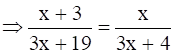(x + 3) (3x + 4) = x (3x + 19)

3x2 + 4x + 9x + 12 = 3x2 + 19x

19x – 13x = 12

6x = 12

∴ x = 12/6 = 23. In figure, if ∠1 =∠2 and ΔNSQ = ΔMTR, then prove that ΔPTS ~ ΔPRQ.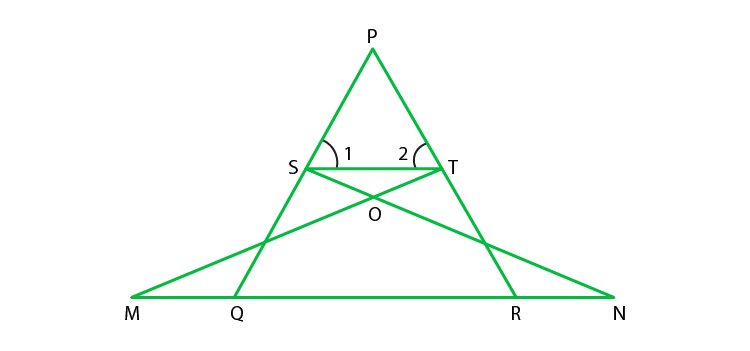Solution:

According to the question,

∆ NSQ ≅ ∆MTR

∠1 = ∠2

Since,

∆NSQ = ∆MTR

So,

SQ = TR ….(i)

Also,

∠1 = ∠2 ⇒ PT = PS….(ii)

[Since, sides opposite to equal angles are also equal]

From Equation (i) and (ii).

PS/SQ = PT/TR

⇒ ST || QR

By converse of basic proportionality theorem, If a line is drawn parallel to one side of a triangle to intersect the other sides in distinct points, the other two sides are divided in the same ratio.

∴ ∠1 = PQR

And

∠2 = ∠PRQ

In ∆PTS and ∆PRQ.

∠P = ∠P [Common angles]

∠1 = ∠PQR (proved)

∠2 = ∠PRQ (proved)

∴ ∆PTS – ∆PRQ

[By AAA similarity criteria]

Hence proved.

4. Diagonals of a trapezium PQRS intersect each other at the point 0, PQ || RS and PQ = 3 RS. Find the ratio of the areas of Δ POQ and Δ ROS.

Solution:

According to the question,

PQRS is a trapezium in which PQ‖RS and PQ = 3RS

PQ/RS = 3/1 = 3 …(i)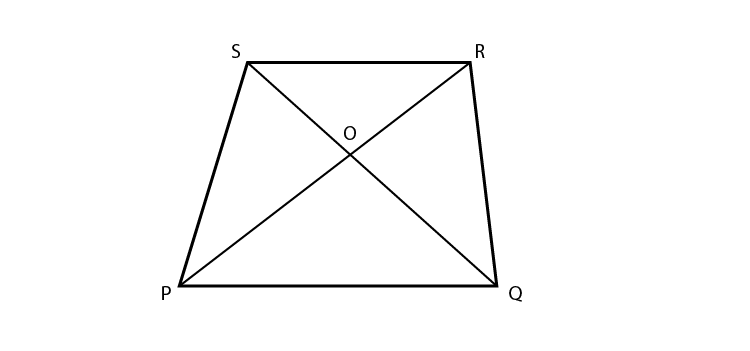In ∆POQ and ∆ROS,

∠SOR = ∠QOP [vertically opposite angles]

∠SRP = ∠RPQ [alternate angles]

∴ ∆POQ ∼ ∆ROS [by AAA similarity criterion]

By property of area of similar triangle,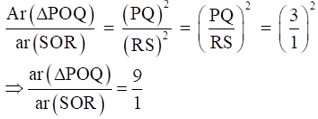Therefore, the required ratio = 9:1.

5. In figure, if AB || DC and AC, PQ intersect each other at the point O. Prove that OA.CQ = 0C.AP.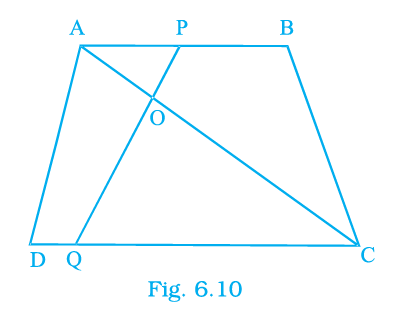Solution:

According to the question,

AC and PQ intersect each other at the point O and AB||DC.

From ∆AOP and ∆COQ,

∠AOP = ∠COQ [Since they are vertically opposite angles]

∠APO = ∠CQO [since, AB||DC and PQ is transversal, the angles are alternate angles]

∴ ∆AOP ∼ ∆COQ [using AAA similarity criterion]

Then, since, corresponding sides are proportional

We have,

OA/OC = AP/CQ

OA × CQ = OC × AP

Hence Proved.

6. Find the altitude of an equilateral triangle of side 8 cm.

Solution:

Let ABC be an equilateral triangle of side 8 cm

AB = BC = CA = 8 cm. (all sides of an equilateral triangle is equal)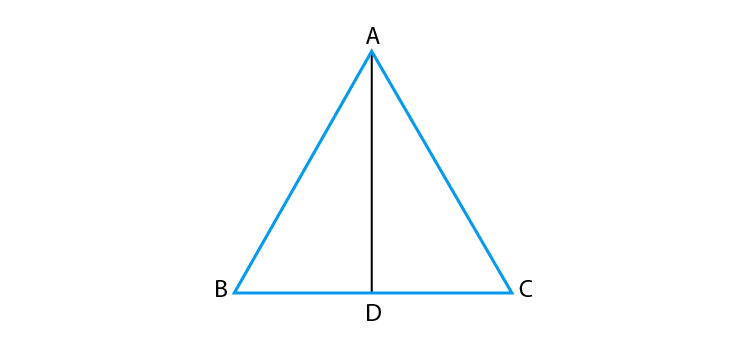Draw altitude AD which is perpendicular to BC.

Then, D is the mid-point of BC.

∴ BD = CD = ½

BC = 8/2 = 4 cm

Now, by Pythagoras theorem

⇒ (8)2 = AD2 + (4)2

⇒ 64 = AD2 + 16

⇒ AD = √48 = 4√3 cm.

Hence, altitude of an equilateral triangle is 4√3 cm.

7. If ∆ABC ∼ ∆DEF, AB = 4 cm, DE = 6, EF = 9 cm and FD = 12 cm, then find the perimeter of ∆ABC.

Solution:

According to the question,

AB = 4 cm,

DE = 6 cm

EF = 9 cm

FD = 12 cm

Also,

∆ABC ∼ ∆DEF

We have,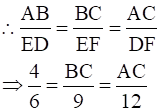By taking first two terms, we have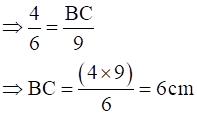And by taking last two terms, we have,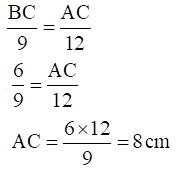Now,

Perimeter of ∆ABC = AB + BC + AC

= 4 + 6 + 8 = 18 cm

Thus, the perimeter of the triangle is 18 cm.

## Exercise 6.4

1. In Fig. 6.16, if ∠A = ∠C, AB = 6 cm, BP = 15 cm, AP = 12 cm and CP = 4 cm, then find the lengths of PD and CD.Solution:

According to the question,

∠A = ∠C,

AB = 6 cm, BP = 15 cm,

AP = 12 cm

CP = 4 cm

From ∆APB and ∆CPD,

∠A = ∠C

∠APB = ∠CPD [vertically opposite angles]

∴ By AAA similarity criteria,

∆APD ∼ ∆CPD

Using basic proportionality theorem,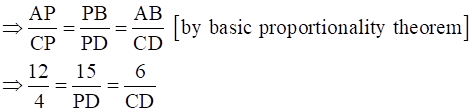Considering AP/CP = PB/PD, we get,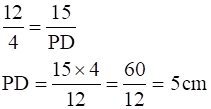Considering, AP/CP = AB/CD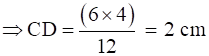Therefore,

Length of PD = 5 cm

Length of CD = 2 cm

2. It is given that ∆ ABC ~ ∆ EDF such that AB = 5 cm, AC = 7 cm, DF= 15 cm and DE = 12 cm. Find the lengths of the remaining sides of the triangles.

Solution: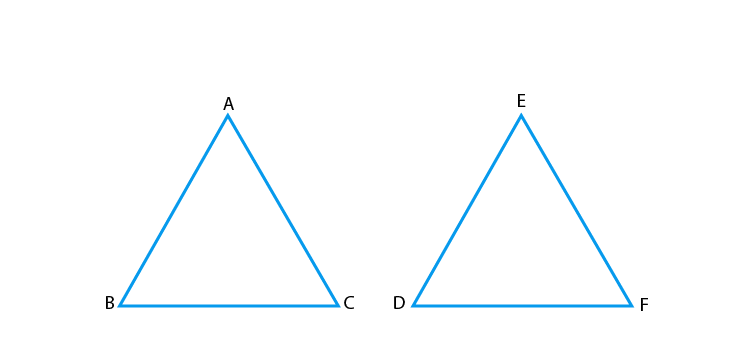According to the question,

∆ABC ∼ ∆EDF

From property of similar triangle,

We know that, corresponding sides of ∆ABC and ∆EDF are in the same ratio.

AB/ED = AC/EF = BC/DF ….(i)

According to the question,

AB = 5cm, AC = 7cm

DF = 15cm and DE = 12cm

Substituting these values in Equation (i), we get,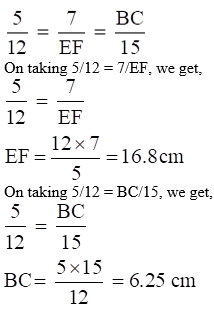Hence, lengths of the remaining sides of the triangles are EF = 16.8 cm and BC = 6.25 cm

3. Prove that if a line is drawn parallel to one side of a triangle to intersect the other two sides, then the two sides are divided in the same ratio.

Solution:

Let a ∆ABC in which a line DE parallel to BC intersects AB at D and AC at E.

To prove DE divides the two sides in the same ratio.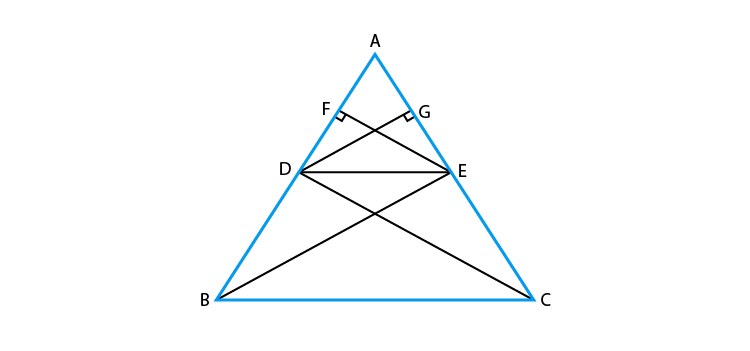Construction:

Join BE, CD

Draw EF ⊥ AB and DG ⊥ AC.

We know that,

Area of triangle = ½ × base × height

Then,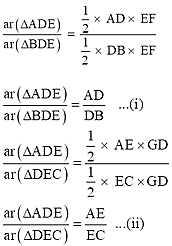Since,

∆BDE and ∆DEC lie between the same parallel DE and BC and are on the same base DE.

We have,

area (∆BDE) = area(∆DEC) …..(iii)

From Equation (i), (ii) and (iii),

We get,

Hence proved.

4. In Fig 6.17, if PQRS is a parallelogram and AB||PS, then prove that OC||SR.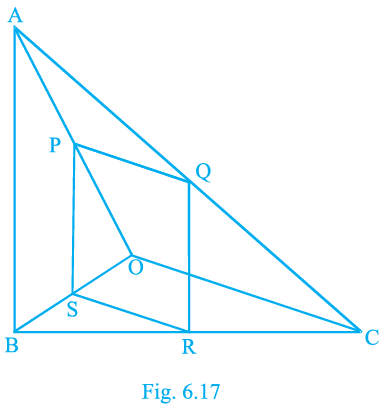Solution:

According to the question,

PQRS is a parallelogram,

Therefore, PQ || SR and PS || QR.

Also given, AB || PS.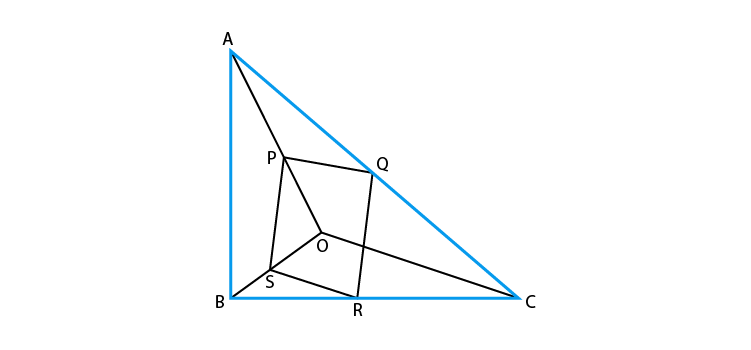To prove:

OC || SR

From ∆OPS and OAB,

PS||AB

∠POS = ∠AOB [common angle]

∠OSP = ∠OBA [corresponding angles]

∆OPS ∼ ∆OAB [by AAA similarity criteria]

Then,

Using basic proportionality theorem,

We get,

PS/AB = OS/OB …(i)

From ∆CQR and ∆CAB,

QR || PS || AB

∠QCR = ∠ACB [common angle]

∠CRQ = ∠CBA [corresponding angles]

∆CQR ∼ ∆CAB

Then, by basic proportionality theorem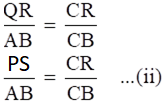[PS ≅ QR Since, PQRS is a parallelogram,]

From Equation (i) and (ii),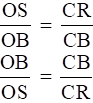Subtracting 1 from L.H.S and R.H.S, we get,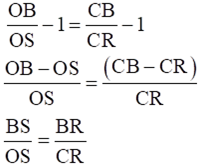SR || OC [By converse of basic proportionality theorem]

Hence proved.

5. A 5 m long ladder is placed leaning towards a vertical wall such that it reaches the wall at a point 4 m high. If the foot of the ladder is moved 1.6 m towards the wall, then find the distance by which the top of the ladder would slide upwards on the wall.

Solution:

Let the length of the ladder = AC = 5 m

Let the height of the wall on which ladder is placed = BC = 4m.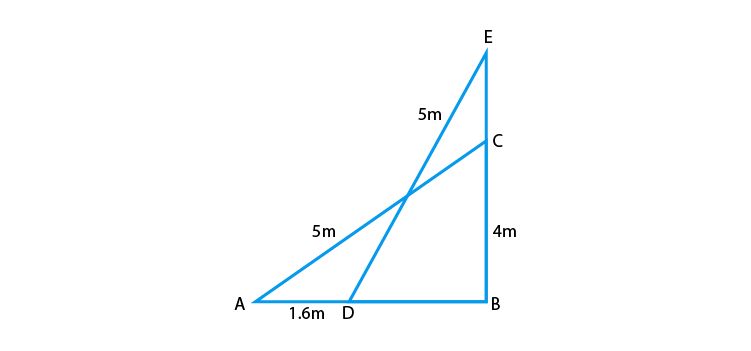From right angled ∆EBD,

Using the Pythagoras Theorem,

ED2 = EB2 + BD2

(5)2 = (EB)2 + (14)2 [ BD = 1.4]

25 = (EB)2 + 1.96

(EB)2 = 25 –1.96 = 23.04

EB = √23.04 = 4.8

Now, we have,

EC = EB – BC = 4.8 – 4 = 0.8

Hence, the top of the ladder would slide upwards on the wall by a distance of 0.8 m.

6. For going to a city B from city A, there is a route via city C such that AC⊥CB, AC = 2 x km and CB = 2 (x + 7) km. It is proposed to construct a 26 km highway which directly connects the two cities A and B. Find how much distance will be saved in reaching city B from city A after the construction of the highway.

Solution:

According to the question,

AC⊥CB,

AC = 2x km,

CB = 2 (x + 7) km and AB = 26 km

Thus, we get ∆ ACB right angled at C.

Now, from ∆ACB,

Using Pythagoras Theorem,

AB2 = AC2 + BC2

⇒ (26)2 = (2x)2 + {2(x + 7)}2

⇒ 676 = 4x2 + 4(x2 + 196 + 14x)

⇒ 676 = 4x2 + 4x2 + 196 + 56x

⇒ 676 = 82 + 56x + 196

⇒ 8x2 + 56x – 480 = 0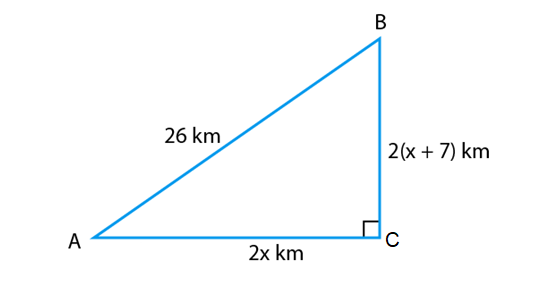Dividing the equation by 8, we get,

x2 + 7x – 60 = 0

x2 + 12x – 5x – 60 = 0

x(x + 12)-5(x + 12) = 0

(x + 12)(x – 5) = 0

∴ x = -12 or x = 5

Since the distance can’t be negative, we neglect x = -12

∴ x = 5

Now,

AC = 2x = 10km

BC = 2(x + 7) = 2(5 + 7) = 24 km

Thus, the distance covered to city B from city A via city C = AC + BC

AC + BC = 10 + 24

= 34 km

Distance covered to city B from city A after the highway was constructed = BA = 26 km

Therefore, the distance saved = 34 – 26 = 8 km.

7. A flag pole 18 m high casts a shadow 9.6 m long. Find the distance of the top of the pole from the far end of the shadow.

Solution: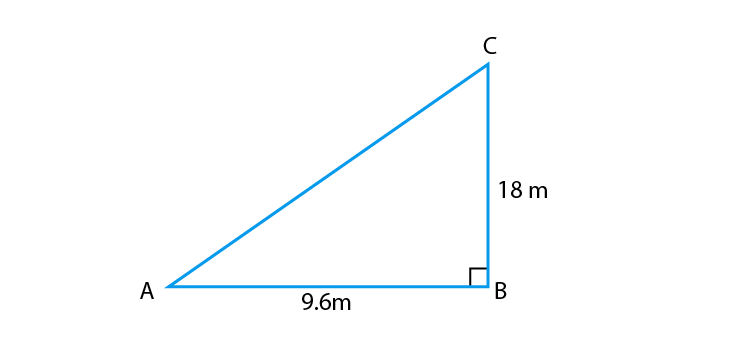Let MN = 18 m be the flag pole and its shadow be LM = 9.6 m.

The distance of the top of the pole, N from the far end, L of the shadow is LN.

In right angled ∆LMN,

LN2 = LM2 + MN2 [by Pythagoras theorem]

⇒ LN2 = (9.6)2 + (18)2

⇒ LN2 = 9.216 + 324

⇒ LN2 = 416.16

∴ LN = √416.16 = 20.4 m

Hence, the required distance is 20.4 m

To help students master the chapter concepts easily, we are offering free NCERT Exemplar for Class 10 Maths Chapter 6 – triangles PDF here, which can be easily downloaded. Also, they can make use of exemplar books, notes, question papers, and 10th Class Maths NCERT Solutions to have a thorough practice for each chapter.

Students are advised to check the sample papers and previous year questions and solve them to get an idea of questions asked from the chapter, Triangles in Class 10th board exams. Also, practice 10th standard Exemplar Solutions for Maths all chapters here. Download BYJU’S -The Learning App and get personalized videos to learn about concepts such as Triangles.

## Frequently Asked Questions on NCERT Exemplar Solutions for Class 10 Maths Chapter 6

### Give me an overview of Chapter 6 of NCERT Exemplar Solutions for Class 10 Maths?

This chapter has an overall of five topics/ subtopics with answers at the end. Provided below are the list of topics discussed in this chapter.
Similar figures
Triangles and their similarity
Conditions for similarity in triangles
Areas of similar triangles and their ratios
Triangle theorems and proofs based on Pythagoras theorem

### Why should I download BYJU’S NCERT Exemplar Solutions for Class 10 Maths Chapter 6?

There are numerous reasons why you should download the NCERT Solutions Exemplar for Class 10 Maths Chapter 6 designed by the experts at BYJU’S.
1. The CBSE board recommends the students to refer to the NCERT Exemplar textbooks as it is one of the best study materials from the exam point of view.
2. NCERT exemplar Solutions plays a significant role as the answers to all the questions present in the NCERT textbook are available in one place.
3. The subject matter experts and faculty at BYJU’S provide solutions in a concise manner to help students score well in the exam.

### What is the Pythagorean theorem according to NCERT Exemplar Solutions for Class 10 Maths Chapter 6?

Pythagoras theorem states that “In a right-angled triangle, the square of the hypotenuse side is equal to the sum of squares of the other two sides“. The sides of this triangle have been named as Perpendicular, Base and Hypotenuse. Here, the hypotenuse is the longest side, as it is opposite to the angle 90°. The sides of a right triangle (say a, b and c) which have positive integer values, when squared, are put into an equation, also called a Pythagorean triple.